# Current Electricity

## Exercise 8(A)

#### Question 1

Define the term current and state it's S.I. unit.

Current is defined as the rate of flow of charge. The S.I. unit of charge is coulomb and therefore current is measured in coulomb per second which has been given the name ampere (A).

#### Question 2

Define the term electric potential. State it's S.I. unit.

The electric potential at a point is defined as the amount of work done per unit charge in bringing a positive test charge from infinity to that point. The S.I. unit of electric potential is volt.

#### Question 3

How is the electric potential difference between the two points defined? State it's S.I. unit.

The potential difference (p.d.) between two points is equal to the work done per unit charge in moving a positive test charge from one point to the other. It's S.I. unit is volt. It is a scalar quantity.

#### Question 4

Explain the statement 'the potential difference between two points is 1 volt'.

The potential difference between two points is said to be 1 volt if the work done in moving 1 coulomb charge from one point to other is 1 joule i.e.,

1 volt = $\dfrac{\text{1 joule}}{\text{1 coulomb}}$ = 1 J C-1

#### Question 5

(a) State whether the current is a scalar or vector? What does the direction of current convey?

(b) State whether the potential is a scalar or vector? What does the positive and negative sign of potential convey?

(a) Current is a scalar quantity.

By stating the direction of current in a conductor we mean that the direction of motion of electrons is opposite to it.

(b) Potential is a scalar quantity.

When the potential is positive at a point in the vicinity of a positive charge since work has to be done on the positive test charge against the repulsive force due to the positive charge in bringing it from infinity, while it is negative at a point in the vicinity of a negative charge since the attractive force on test charge due to the negative charge does work by itself.

#### Question 6

Define the term resistance. State it's S.I. unit.

The obstruction offered to the flow of current by the conductor (or wire) is called it's resistance.

The S.I. unit of resistance is Ohm.

#### Question 7

(a) Name the particles which are responsible for the flow of current in a metallic wire.

(b) Explain the flow of current in a metallic wire on the basis of movement of the particles named by you above in part (a).

(c) What is the cause of resistance offered by the metallic wire in the flow of current through it?

(a) The particles which are responsible for the flow of current in a metallic wire are free electrons.

(b) In a metallic wire, the moving charges are the free electrons which constitute the current.

If n electrons pass through the cross section of a conductor in time t, then total charge passed through the conductor is given as

Q (charge) = n × e

and the current in conductor is

$\text{I} = \dfrac{\text{Q}}{\text{t}} = \dfrac{\text{ne}}{\text{t}}$

(c) When the ends of a metal wire are connected to a cell, the electrons inside it experience a force in direction from the end at negative potential to positive, due to which they began to move as shown in the diagram below.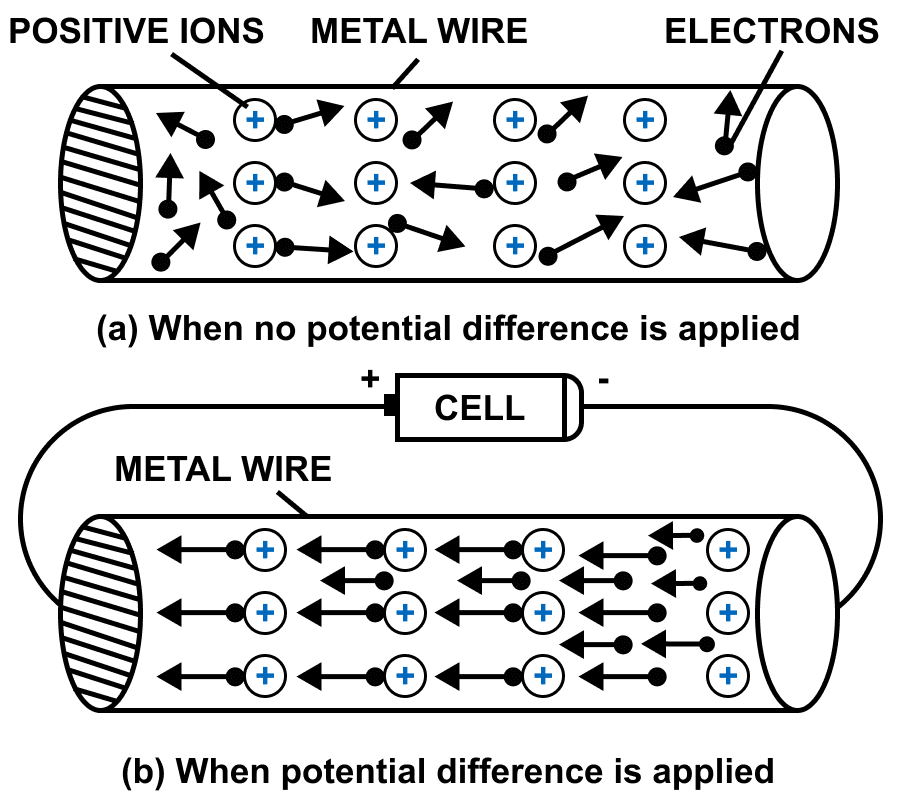Due to force, their speed increases but during the movement they collide with the fixed positive ions and loose some of their kinetic energy due to which their speed decreases. This lost energy heats up the wire.

After the collision, they are again accelerated towards the positive potential due to the existing potential difference so their speeds again increases and then again in collision with the positive ions, their speed decreases. This process continues. As a result, the electrons do not move in bulk with a continuously increasing speed, but their is a drift of electrons towards the positive terminal. Thus, a metal wire offers some resistance to the flow of electrons through it.

#### Question 8

State Ohm's law and draw a neat labelled circuit diagram containing a battery, a key, a voltmeter, an ammeter, a rheostat and an unknown resistance to verify it.

According to Ohm's law, the current flowing in a conductor is directly proportional to the potential difference applied across it's ends provided that the physical conditions and the temperature of the conductor remain constant.

If a current I flows in a conductor when the potential difference across it's ends is V and R is the resistance, then according to Ohm's law V = IR

Below circuit diagram shows the setup for verifying Ohm's law: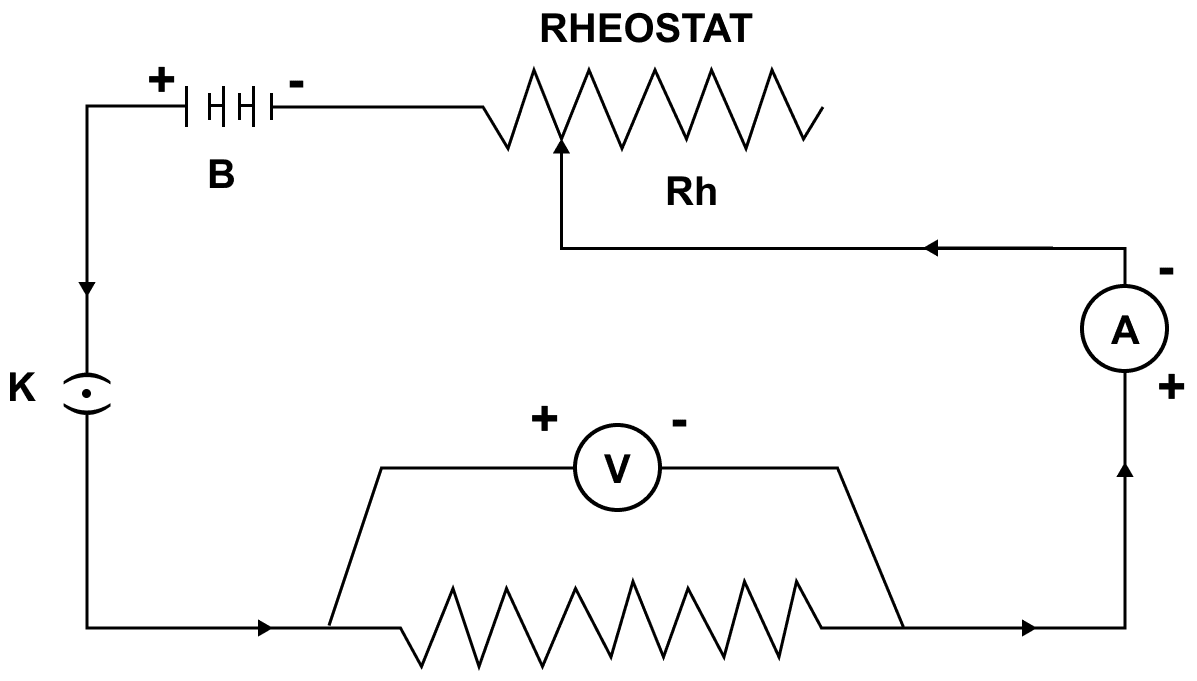#### Question 9

(a) Name and state the law which relates the potential difference and current in a conductor.

(b) What is the necessary condition for a conductor to obey the law named above in part (a)?

(a) The law which relates the potential difference and current in a conductor is known as Ohm's law.

Ohm's law states that the current flowing through the conductor is directly proportional to the potential difference across it's ends provided that the physical conditions and the temperature of the conductor remain constant.

(b) The necessary condition for a conductor to obey Ohm's law is that the physical conditions and the temperature should remain constant.

#### Question 10

(a) Draw a V-I graph for a conductor obeying Ohm's law.

(b) What does the slope of V-I graph for a conductor represent?

(a) V-I graph for a conductor obeying Ohm's law is shown below: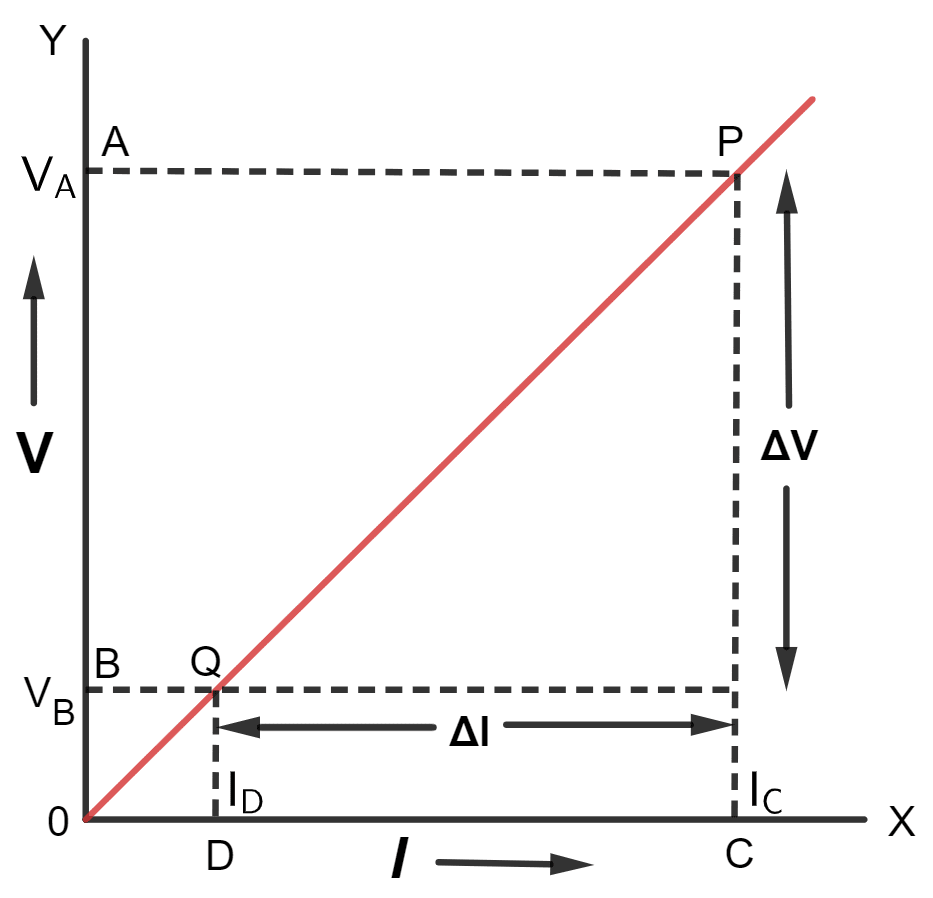(b) Slope of V-I graph for a conductor represents resistance.

#### Question 11

Draw an I-V graph for a linear resistor. What does it's slope represent?

I-V graph for a linear resistor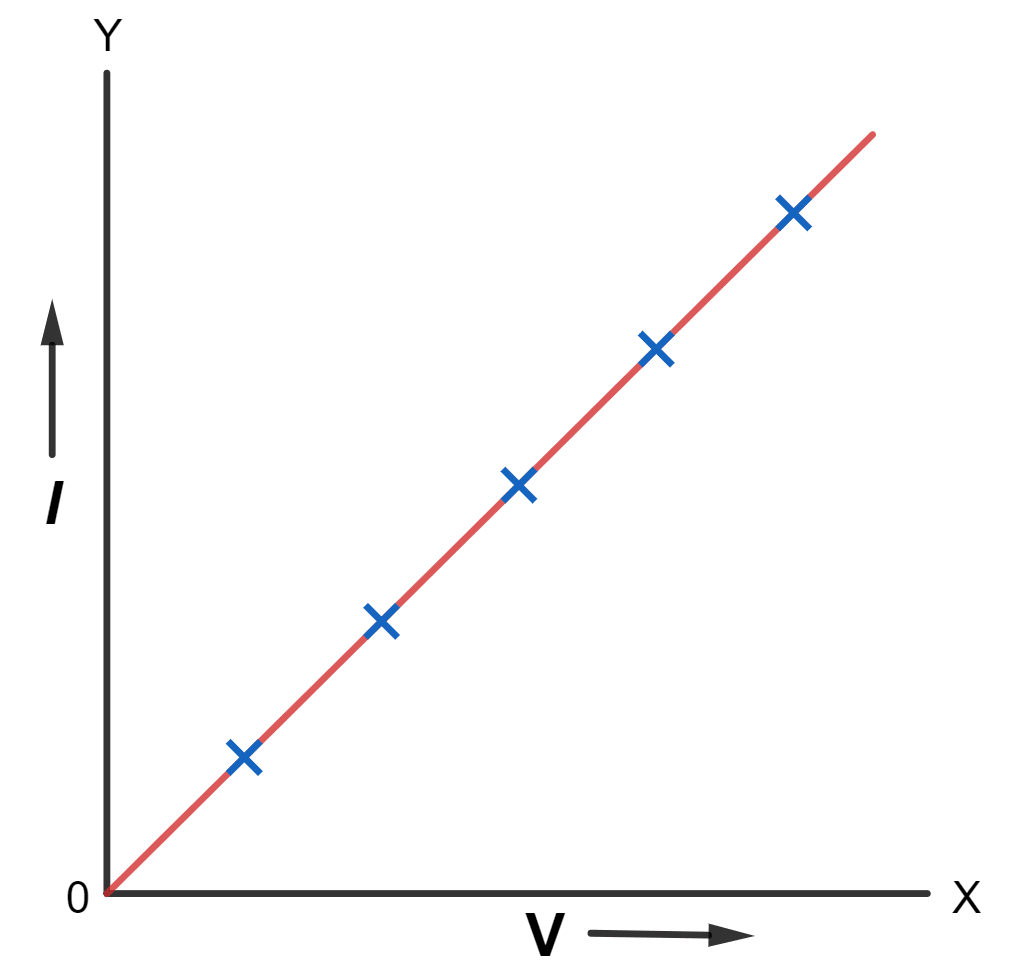Slope of I-V graph — The slope of I-V graph is $\dfrac{ΔI}{ΔV}$ which is the reciprocal of resistance of the conductor i.e.,

Slope = $\dfrac{ΔI}{ΔV}$ = $\dfrac{1}{\text{resistance of the conductor}}$

#### Question 12

What is an ohmic resistor? Give one example of an ohmic resistor. Draw a graph to show it's current–voltage relationship. How is the resistance of the resistor determined from this graph?

The conductors which obey the Ohm's law are called the ohmic resistors or linear resistances. Examples are all metallic conductors (such as Silver, Aluminium, Copper, Iron etc.)

Current–Voltage relationship of an ohmic resistor is shown in the below graph:Resistance is determined in the form of slope from the graph.

#### Question 13

What are non-ohmic resistors? Give one example and draw a graph to show it's current-voltage relationship.

The conductors which do not obey the Ohm's law are known as the non-ohmic resistors (or non-linear resistances). Examples — LED, solar cell, junction diode, etc.

Current-Voltage relationship of non-ohmic resistors is shown in the graph below: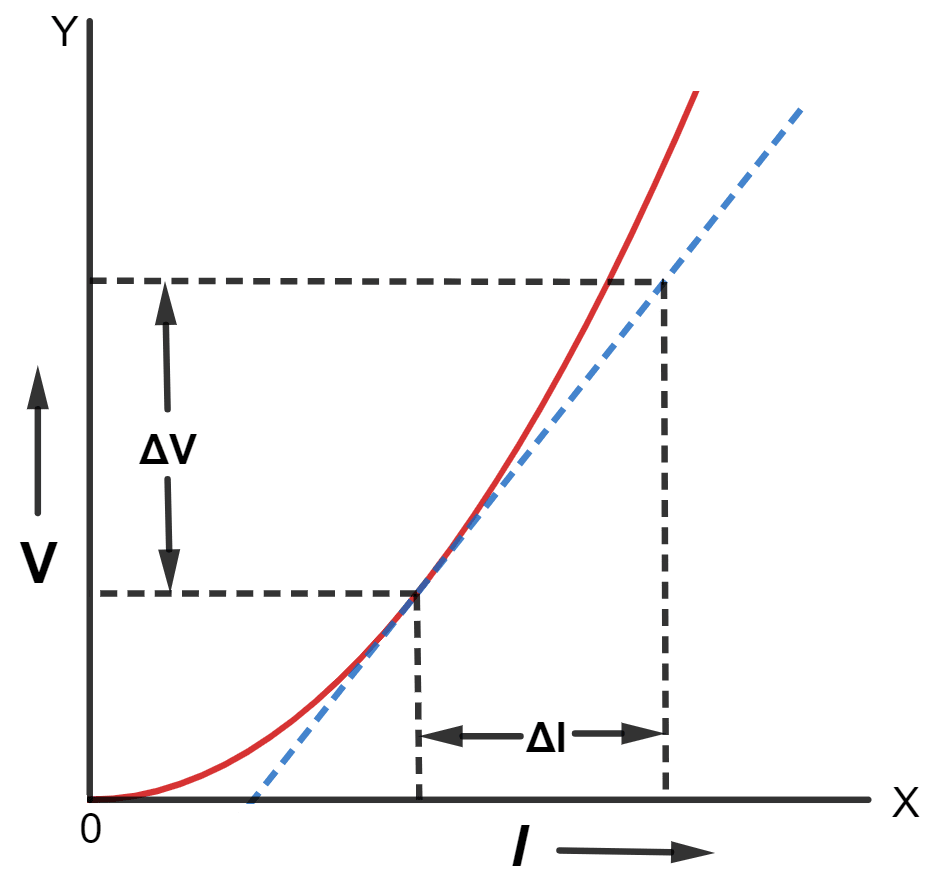#### Question 14

Give two differences between an ohmic and non-ohmic resistor.

Ohmic resistorNon-ohmic resistor
It obeys the Ohm's law i.e., $\dfrac{V}{I}$ is constant for all values of V and I.It does not obey the Ohm's law i.e., $\dfrac{V}{I}$ is not same for all values of V and I.
The graph for potential difference V versus current I is a straight line passing through the origin.The graph for potential difference V versus current I is not a straight line, but is a curve which may not pass through the origin.

#### Question 15

Figure below shows the I-V curves for two resistors. Identify the ohmic and non-ohmic resistors. Give a reason for your answer.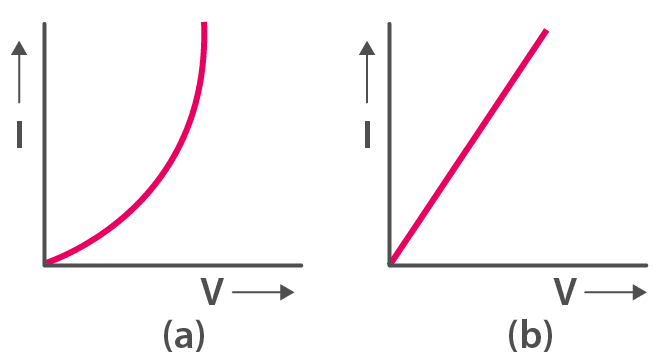Graph (a) is non-ohmic resistor and

Graph (b) is ohmic resistor.

The I-V graph for (b) is a straight line or linear while for (a), the graph is a curve.

#### Question 16

Draw a V-I graph for a conductor at two different temperatures. What conclusion do you draw from your graph for the variation of resistance of conductor with temperature?

V-I graph of a conductor at two different temperatures T1 and T2 is shown below: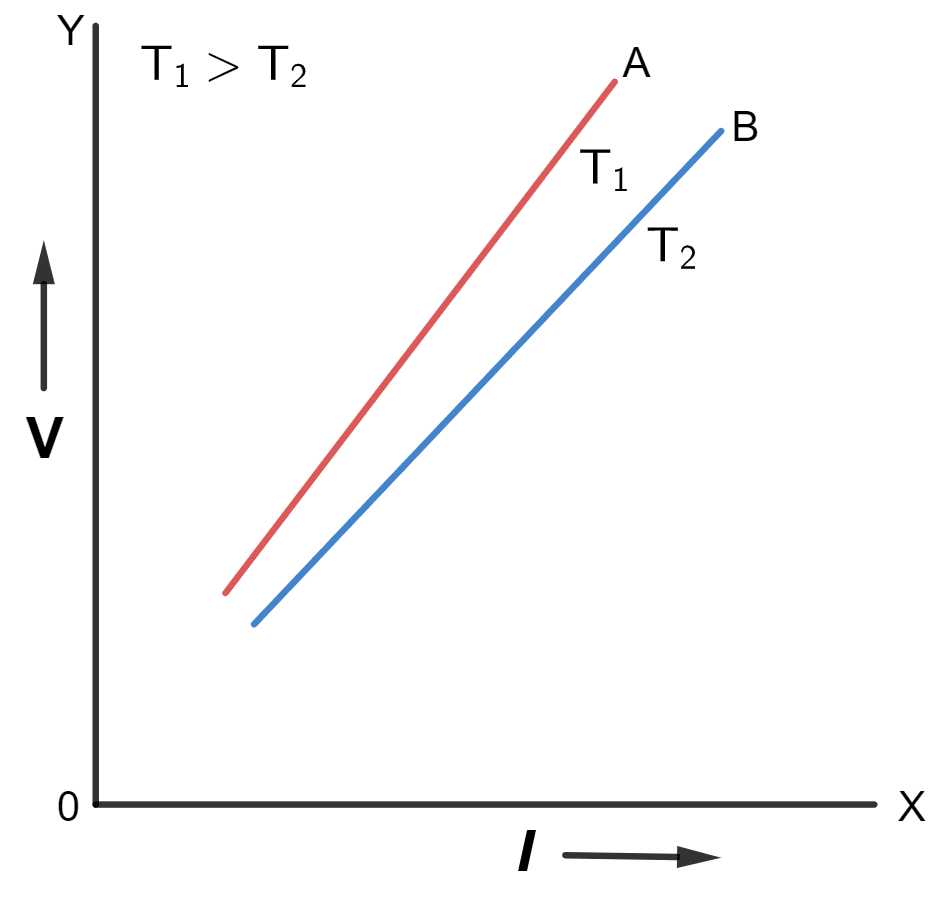Figure shows two straight lines A and B on the V-I graph for a conductor at two different temperatures T1 and T2 (T1 > T2) respectively.

The straight line A is more steeper than the line B because the resistance of conductor is more at high temperature T1 than at low temperature T2

#### Question 17

(a) How does the resistance of a wire depend on it's radius? Explain your answer.

(b) Two copper wires are of same length, but one is thicker than the other. Which will have more resistance?

(a) In a thick conductor, electrons get a larger area of cross section to flow as compared to a thin conductor, therefore a thick wire offers less resistance. The resistance of a conductor is inversely proportional to it's area of cross section (a), normal to the direction of flow of current i.e.,

R ∝ $\dfrac{1}{a}$

Hence,

R ∝ $\dfrac{1}{πr^2}$

where r is the radius of the wire.

Hence, resistance of a wire is inversely proportional to the square of the radius of the wire.

(b) As we have seen above that the resistance of a conductor is inversely proportional to it's area of cross section (a), normal to the direction of flow of current. Hence, the thin wire, will offer more resistance as it's area of cross section is less.

#### Question 18

How does the resistance of a wire depend on it's length ? Give a reason for your answer.

Resistance of a wire is directly proportional to the length of the wire.

R ∝ l

In a long conductor, the number of collisions of free electrons with the positive ions will be more as compared to a shorter one. Therefore, a longer conductor offers more resistance. Hence, the resistance of a conductor is directly proportional to the length of the wire.

#### Question 19

How does the resistance of a metallic wire depend on it's temperature? Explain with reason.

With the increase in temperature of a conductor, the random motion of electrons increases. As a result, the number of collisions of electrons with the positive ions increase. Hence, the resistance of a conductor increases with an increase in it's temperature.

#### Question 20

Two wires, one of copper and other of iron, are of the same length and same radius. Which will have more resistance? Give reason.

The iron wire will have more resistance.

Iron wire has more resistance as compared to copper because specific resistance of iron is more than that of copper.

#### Question 21

Name three factors on which resistance of a given wire depends and state how it is affected by the factors stated by you?

The three factors on which the resistance of wire depends are —

1. Dependence on length of the wire — the resistance of a wire is directly proportional to the length of the wire.
R ∝ l
2. Dependence on the thickness of the wire — the resistance of a wire is inversely proportional to it's area of cross section (a) normal to the direction of flow of current.
R ∝ $\bold{\dfrac{1}{a}}$
3. Dependence on the temperature of the wire — the resistance of conductor increases with an increase in it's temperature.

#### Question 22

Define the term specific resistance and state it's S.I. unit.

Specific resistance of a material is the resistance of a wire of that material of unit length and unit area of cross section.

The S.I. unit of specific resistance is ohm × metre or (Ω m)

#### Question 23

Write an expression connecting the resistance of a wire and specific resistance of it's material. State the meaning of symbols used.

The expression is —

R = ρ $\dfrac{ l}{a}$

where

R = resistance of wire
ρ = specific resistance of the material of wire
l = length of wire
a = area of cross section of wire

#### Question 24

State the order of specific resistance of (i) a metal, (ii) a semiconductor and (iii) an insulator.

The order of specific resistance is as follows:

1. The specific resistance is very low for metals, because it allows most of current to pass through it.
2. The specific resistance is low for semiconductor.
3. The specific resistance is very high for insulators, as the current won't pass through it.

#### Question 25

(a) Name two factors on which the specific resistance of a wire depends?

(b) Two wires A and B are made of copper. The wire A is long and thin while the wire B is short and thick. Which will have more specific resistance?

(a) Two factors on which the specific resistance of a wire depends are —

1. Material of the substance — It is a characteristic property of the substance. It is different for different substances.
2. Temperature of the substance — It increases with the increase in temperature for metals, but it decreases with the increase in temperature for the semiconductor.

(b) Both the wires will have the same specific resistance because the specific resistance is a characteristic property of the material and as both the wires are of copper hence both will have same specific resistance.

#### Question 26

Name a substance of which the specific resistance remains almost unchanged by the increase in temperature.

The substance whose specific resistance remains almost unchanged with increase in temperature is manganin.

#### Question 27

How does the specific resistance of a semi-conductor change with the increase in temperature?

The specific resistance of a semi-conductor decreases with the increase in temperature.

#### Question 28

How does (a) resistance, and (b) specific resistance of a wire depend on it's (i) length, and (ii) radius?

(a) Dependency of resistance of a wire is as follows:

1. Resistance of a wire is directly proportional to length of the wire.
2. Resistance of a wire is inversely proportional to the square of radius of the wire.

(b) Dependency of specific resistance of a wire is as follows:

1. Specific resistance of a wire does not depend on length of the wire as it is a characteristic property of the material of wire.
2. Specific resistance of a wire does not depend on radius of the wire as it is a characteristic property of the material of wire.

#### Question 29

(a) Name the material used for making connection wires. Give reason for your answer.

(b) Why should a connection wire be thick?

(a) Copper or aluminium materials are used for making connection wires because their specific resistance is very low and hence they possess least possible resistance.

Due to low (or negligible) resistance of connection wires, the current in circuit remains unaffected, and the loss of energy due to heating is prevented. Hence, they are made of materials such as copper or aluminium, whose specific resistance is very small.

(b) The connection wires are made thick so that their resistance becomes low.

#### Question 30

Name a material which is used for making the standard resistor. Give a reason.

Standard resistors are made of magnanin, constantan etc. for which the specific resistance is high and the effect of change in temperature on their resistance is negligible.

#### Question 31

Name the material used for making fuse wire. Give a reason.

The material used for making fuse wire is an alloy of lead and tin because it's melting point is low and it's specific resistance is more than that of copper or aluminium so that the resistance of a short and thin fuse wire is more than that of the connecting wire. It permits current upto it's safe limit to pass through it, but an excessive current melts it so that it blows off and the circuit is broken.

#### Question 32

Name the material used for (i) filament of an electric bulb and (ii) heating element of a room heater.

(i) The material used for the filament of an electric bulb is a tungsten wire because it has a high melting point.

(ii) The material used for the heating element of a room heater is nichrome because the specific resistance of nichrome is high and it's resistance increases to a great extent with the increase in temperature.

#### Question 33

What is a superconductor ? Give one example of it.

A superconductor is a substance of zero resistance (or infinite conductance) at a very low temperature.

Example — Mercury below 4.2 K, lead below 7.25 K and niobium below 9.2 K

Resistances of these substances decrease tremendously with the decrease in temperature and become almost zero in the low temperature range near absolute zero. Zero resistance of a superconductor means it's infinite conductivity (i.e., once a current starts flowing in a superconductor, it persists even when there is no potential across it).

#### Question 34

A substance has zero resistance below 1 K. What is such a substance called ?

A substance of zero resistance (or infinite conductance) at a very low temperature is called a superconductor.

## Multiple Choice Type

#### Question 1

Which of the following is an ohmic resistance?

1. LED
2. junction diode
3. filament of a bulb
4. nichrome wire ✓

The conductors which obey the Ohm's law are called the ohmic resistors or linear resistances.

A Nichrome wire obeys Ohm's law hence it is an ohmic resistor.

#### Question 2

For which of the following substances, resistance decreases with increase in temperature?

1. copper
2. mercury
3. carbon ✓
4. platinum

Resistance decreases with increase in temperature for carbon.

## Numericals

#### Question 1

In a conductor, 6.25 × 1016 electrons flow from it's end A to B in 2 s. Find the current flowing through the conductor. (e = 1.6 × 10-19 C)

Given,

Number of electrons flowing (n) = 6.25 × 1016

Time taken (t) = 2 s

e = 1.6 × 10-19 C

Current in conductor is given by,

$\text{I} = \dfrac{\text{ne}}{\text{t}}$

Substituting the values in the formula above we get,

$I = \dfrac{6.25 \times 10^{16} \times 1.6 \times 10^{-19}}{2} \\[0.5em] I = \dfrac{6.25 \times 1.6 \times 10^{-3}}{2} \\[0.5em] I = 6.25 \times 0.8 \times 10^{-3} \\[0.5em] I = 5 \times 10^{-3} A$

Hence, the current flowing through the conductor = 5 mA from B to A

#### Question 2

A current of 1.6 mA flows through a conductor. If charge on an electron is -1.6 x 10-19 coulomb, find the number of electrons that will pass each second through the cross section of that conductor.

Given,

Current (I) = 1.6 mA = 1.6 × 10-3 A

Charge of one electron = -1.6 × 10-19 coulomb

t = 1 s

Charge flowing through the conductor in one second

Charge (Q) = current (I) x time (t)

Substituting the values in the formula above we get,

Q = 1.6 x 10-3 x 1 = 1.6 x 10-3

Hence,

$\text{No. of electrons} = \dfrac{\text{Total charge flowing}}{\text{Charge on one electron}}$

$= \dfrac{1.6 \times 10^{-3}}{1.6 \times 10^{-19}} \\[0.5em] = 10^{16} \\[0.5em]$

Therefore, the number of electrons that will pass each second through the cross section of that conductor = 1016

#### Question 3

Find the potential difference required to flow a current of 200 mA in a wire of resistance 20 Ω.

Given,

Current (I) = 200 mA = 0.2 A

Resistance (R) = 20 Ω

Potential difference (V) = ?

From Ohm's law

V = IR

Substituting the values in the formula above, we get,

V = 0.2 × 20

V = 4 V

Hence, the required potential difference = 4 V

#### Question 4

An electric bulb draws 1.2 A current at 6.0 V. Find the resistance of filament of bulb while glowing.

Given,

Current (I) = 1.2 A

Potential difference (V) = 6.0 V

Resistance (R) = ?

From Ohm's law

V = IR

Substituting the values in the formula above, we get,

$6 = 1.2 \times R \\[0.5em] \Rightarrow R = \dfrac{6}{1.2} \\[0.5em] \Rightarrow R = 5 \varOmega$

Hence, resistance of filament of bulb = 5 Ω

#### Question 5

A car bulb connected to a 12 volt battery draws 2 A current when glowing. What is the resistance of the filament of the bulb? Will the resistance be more, same or less when the bulb is not glowing.

Given,

Potential difference (V) = 12 V

Current (I) = 2 A

Resistance = ?

From Ohm's law

V = IR

Substituting the values in the formula above, we get,

$12 = 2 \times R \\[0.5em] \Rightarrow R = \dfrac{12}{2} \\[0.5em] \Rightarrow R = 6 \varOmega$

Hence, the resistance of the filament of the bulb = 6 Ω

The resistance will be less when the bulb is not glowing.

#### Question 6

Calculate the current flowing through a wire of resistance 5 Ω connected to a battery of potential difference 3 V.

Given,

Resistance (R) = 5 Ohm

Potential difference (V) = 3 V

Current (I) = ?

From Ohm's law

V = IR

Substituting the values in the formula above, we get,

$3 = I \times 5 \\[0.5em] \Rightarrow I = \dfrac{3}{5} \\[0.5em] \Rightarrow I = 0.6 A$

Hence, the current flowing through the wire = 6 A

#### Question 7

In an experiment of verification of Ohm's law, following observations are obtained.

Potential difference V (in volt)0.51.01.52.02.5
Current I (in amp)0.20.40.60.81.0

Draw a V-I graph and use this graph to find —

(a) the potential difference V when the current I is 0.5 A.

(b) the current I when the potential difference V is 0.75 V.

(c) the resistance in circuit.

V-I graph for the readings is shown below: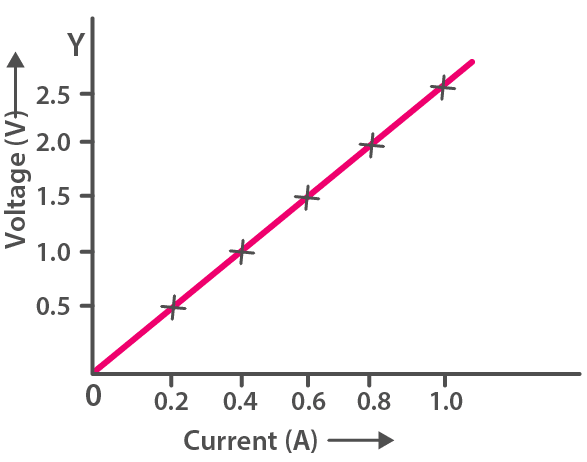(a) We can observe from the graph that when current is 0.5 A then potential difference is 1.25 V.

(b) We can observe from the graph that when potential difference is 0.75 V, then current is 0.3 A.

(c) Slope of the graph gives the resistance, and as the graph is linear

So, if potential difference (V) = 1.0 V

current (I) = 0.4 A

From Ohm's law

V = IR

Substituting the values in the formula above, we get,

$1.0 = 0.4 \times R \\[0.5em] \Rightarrow R = \dfrac{1.0}{0.4} \\[0.5em] \Rightarrow R = \dfrac{10}{4} \\[0.5em] \Rightarrow R = 2.5 \varOmega$

Hence, the resistance in circuit = 2.5 Ω

#### Question 8

Two wires of the same material and same length have radii 1 mm and 2 mm respectively. Compare (i) their resistances (ii) their specific resistance.

(i) R = ρ $\dfrac{l}{a}$ = ρ $\dfrac{l}{πr^2}$

Resistance for wire of radius 1 mm,

R1 = ρ $\dfrac{l}{π1^2}$ = ρ $\dfrac{l}{π}$

Resistance for wire of radius 2 mm,

R2 = ρ $\dfrac{l}{π2^2}$ = ρ $\dfrac{l}{π4}$

Hence, ratio between the two,

R1 : R2

$= \dfrac{ρ\dfrac{l}{π}}{ρ\dfrac{l}{π4}} \\[1em] = \dfrac{4}{1} \\[0.5em] = 4 : 1$

Hence, the ratio of resistance between the two wires = 4 : 1

(ii) The specific resistance of the two wires will be same because the specific resistance is a characteristic property of the material, hence it does not change with radius.

Therefore, ratio of specific resistance between the two wires = 1 : 1

#### Question 9

A given wire of resistance 1 Ω is stretched to double it's length. What will be it's new resistance?

When the wire is stretched to double it's length, it's area of cross section becomes half and it's length becomes double.

Let, a be the area of initial cross section and ρ be the specific resistance of the material of wire.

Then,

length = l,

R = 1 Ω,

new length = 2l,

new area = $\dfrac{a}{2}$

From relation

R = ρ $\dfrac{l}{a}$ = ρ $\dfrac{l}{πr^2}$

Initial resistance R1 = 1 = ρ $\dfrac{l}{a}$    [Equation 1]

New resistance Rn = ρ $\dfrac{2l}{\dfrac{a}{2}}$ = ρ $\dfrac{4l}{a}$    [Equation 2]

On dividing eqn (ii) by (i), we get,

$\dfrac{R_n}{1} = \dfrac{ ρ \dfrac{4l}{a}}{ρ \dfrac{l}{a}} \\[0.5em] \Rightarrow R_n = 4 \varOmega$

Hence, the new resistance = 4 Ω.

#### Question 10

A wire of resistance 3 ohm and length 10 cm is stretched to length 30 cm. Assuming that it has a uniform cross-section, what will be it's new resistance?

Given,

Resistance (R) = 3 Ω

Length (l) = 10 cm

Let, a be the area of initial cross section and ρ be the specific resistance of the material of wire.

Then,

R = 3 Ω

length = 10 cm,

new length = 30 cm,

new area a = $\dfrac{a}{3}$

From relation

R = ρ $\dfrac{l}{a}$ = ρ $\dfrac{l}{πr^2}$

Initial resistance 3 = ρ $\dfrac{10}{a}$    [Equation 1]

New resistance R2 = ρ $\dfrac{30}{\dfrac{a}{3}}$ = ρ $\dfrac{90}{a}$    [Equation 2]

On dividing eqn (i) by (ii), we get,

$\dfrac{3}{R_2} = \dfrac{ρ \dfrac{10}{a}}{ ρ \dfrac{90}{a}} \\[0.5em] \dfrac{3}{R_2} = \dfrac{10}{90} \\[0.5em] \dfrac{3}{R_2} = \dfrac{1}{9} \\[0.5em] \Rightarrow R_2 = 3 \times 9 \\[0.5em] \Rightarrow R_2 = 27 \varOmega \\[0.5em]$

Hence, the new resistance = 27 Ω.

#### Question 11

A wire of resistance 9 Ohm having length 30 cm is tripled on itself. What is it's new resistance?

Given,

Resistance (R) = 9 Ω

When the wire is tripled on itself, it's area of cross section becomes thrice and it's length becomes $\dfrac{l}{3}$.

Let, a be the area of initial cross section and ρ be the specific resistance of the material of wire.

Then,

length = 30 cm,

new length = $\dfrac{l}{3}$ = $\dfrac{30}{3}$ = 10 cm,

new area an = 3a

From relation

R = ρ $\dfrac{l}{a}$

Initial resistance 9 = ρ $\dfrac{30}{a}$    [Equation 1]

New resistance Rn = ρ $\dfrac{10}{3a}$    [Equation 2]

On dividing eqn (ii) by (i), we get,

$\dfrac{R_n}{9} = \dfrac{ρ \dfrac{10}{3a}}{ ρ \dfrac{30}{a}} \\[0.5em] \dfrac{R_n}{9} = {\dfrac{10}{90}} \\[0.5em] \dfrac{R_n}{9} = {\dfrac{1}{9}} \\[0.5em] R_n = 1 \varOmega \\[0.5em]$

Hence, the new resistance = 1 Ω.

#### Question 12

What length of copper wire of specific resistance 1.7 x 10-8 Ω m and radius 1 mm is required so that it's resistance is 1 Ω.

Given,

Specific resistance ρ = 1.7 × 10-8ohm m

Radius r = 1 mm = 10-3m

Resistance R = 1 Ω

Length l = ?

From relation

R = ρ $\dfrac{l}{a}$ = ρ $\dfrac{l}{πr^2}$

Substituting the values in the formula above we get,

$1 = 1.7 \times 10^{-8} \dfrac{l}{π(10^{-3})^2} \\[0.5em] 1 = 1.7 \times 10^{-8} \dfrac{l}{3.14 \times (10^{-3})^2} \\[0.5em] 1 = \dfrac{1.7 \times 10^{-2} \times l}{3.14} \\[0.5em] 1 = \dfrac{1.7 \times l}{314} \\[0.5em] \Rightarrow l = \dfrac{314}{1.7} \\[0.5em] \Rightarrow l = 184.7 m \\[0.5em]$

Hence, the length of the copper wire = 184.7 m.

#### Question 13

The filament of a bulb takes a current 100 mA when potential difference across it is 0.2 V. When the potential difference across it becomes 1.0 V, the current becomes 400 mA. Calculate the resistance of filament in each case and account for the difference.

Case 1 —

Current (I) = 100 m A = 0.1 A

Potential Difference (V) = 0.2 V

From Ohm's law

V = IR

Substituting the values in the formula above, we get,

$0.2 = 0.1 \times R \\[0.5em] \Rightarrow R = \dfrac{0.2}{0.1} \\[0.5em] \Rightarrow R = 2 \varOmega \\[0.5em]$

Hence, resistance of filament of bulb = 2 Ω

Case 2 —

Current (I) = 400 m A = 0.4 A

Potentia Difference (V) = 1.0 V

From Ohm's law

V = IR

Substituting the values in the formula above, we get,

$1.0 = 0.4 \times R \\[0.5em] \Rightarrow R = \dfrac{1.0}{0.4} \\[0.5em] \Rightarrow R = 2.5 \varOmega \\[0.5em]$

Hence, resistance of filament of bulb = 2.5 Ω

Therefore, we observe that with increase in temperature resistance of the wire increases.

Hence, resistance of filament increases with the increase in temperature.

## Exercise 8(B)

#### Question 1

Explain the meaning of the terms e.m.f., terminal voltage and internal resistance of a cell.

e.m.f — e.m.f of a cell is defined as the energy spent (or the work done) per unit charge in taking a positive test charge around the complete circuit of the cell. (i.e., in the circuit outside the cell as well as in the electrolyte inside the cell).

Terminal voltage — The terminal voltage of a cell is defined as the work done per unit charge in carrying a positive test charge around the circuit connected across the terminals of the cell.

Internal resistance — The resistance offered by the electrolyte inside the cell, to the flow of current, is known as the internal resistance of the cell.

#### Question 2

State two differences between the e.m.f. and terminal voltage of a cell.

E.m.f of cellTerminal voltage of cell
It is measured by the amount of work done per unit change in moving a positive test charge in the complete circuit inside and outside the cell.It is measured by the amount of work done per unit charge in moving a positive test charge in the circuit outside the cell.
It is the the characteristic of the cell, i.e., it does not depend on the amount of current drawn from the cell.It depends on the amount of current drawn from the cell. More the current drawn from the cell, less is the terminal voltage.

#### Question 3

Name two factors on which the internal resistance of a cell depends and state how does it depend on the factors stated by you.

The factors on which the internal resistance of a cell depends are —

1. The surface area of the electrodes — larger the surface area of electrodes, less is the internal resistance.
2. The distance between the electrodes — more the distance between the electrodes, greater is the internal resistance.

#### Question 4

A cell of e.m.f. ε and internal resistance r is used to send current to an external resistance R. Write expressions for (a) the total resistance of circuit, (b) the current drawn from the cell, (c) the p.d. across the cell, and (d) voltage drop inside the cell.

Given,

e.m.f. = ε

internal resistance = r

external resistance = R

(a) The total resistance of circuit = R + r

(b) The current drawn from the cell

I = $\dfrac{\text { e.m.f.of cell}}{\text { total resistance}}$ = $\dfrac{\bold{\varepsilon}}{\bold{(R + r)}}$

(c) the p.d. across the cell = V = IR

Substituting from above,

V = $\bold{\dfrac{\varepsilon}{(R + r)}}$ x R

(d) voltage drop inside the cell V = Ir

Substituting from above,

V = $\bold{\dfrac{\varepsilon}{(R + r)}}$ x r

#### Question 5

A cell is used to send current to an external circuit. (a) How does the voltage across it's terminals compare with it's e.m.f. ? (b) Under what condition is the e.m.f. of the cell equal to it's terminal voltage?

(a) When a cell is used to send current to an external circuit, it's terminal voltage V is less than it's e.m.f.

Hence, terminal voltage < e.m.f.

(b) The emf of the cell is equal to it's terminal voltage when no current is drawn.

#### Question 6

Explain why is the p.d. across the terminals of a cell is more in an open circuit and reduced in a closed circuit.

In a closed circuit, the current flows through the circuit. There is a fall of potential across the internal resistance of the cell. Hence, the p.d. across the terminals in a closed circuit is less than the p.d. across the terminals in an open circuit by an amount equal to the potential drop across the internal resistance of the cell.

Hence, the p.d. across the terminals of a cell is more in an open circuit and reduced in a closed circuit.

#### Question 7

Write the expressions for the equivalent resistance R of three resistors R1, R2 and R3 joined in (a) parallel, (b) series.

(a) Equivalent resistance R of three resistors R1, R2 and R3 joined in parallel is given by —

$\dfrac{1}{R}$ = $\dfrac{1}{R}_1$ + $\dfrac{1}{R_2}$ + $\dfrac{1}{R_3}$

(b) Equivalent resistance R of three resistors R1, R2 and R3 joined in series is given by —

R = R1 + R2 + R3

#### Question 8

How would you connect two resistors in series? Draw a diagram. Calculate the total equivalent resistance.

Below diagram shows two resistors connected in series: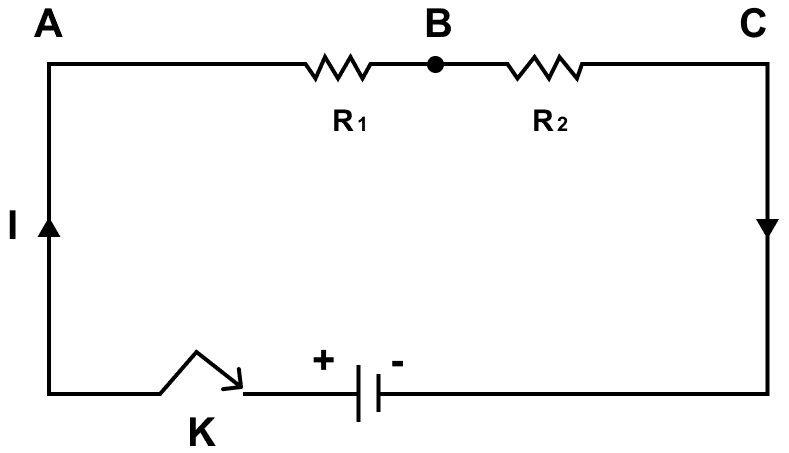If current I is drawn from the battery, the current through each resistor will also be I.

By Ohm's law,

p.d. between A and B is V1 = VA - VB = IR1

p.d. between B and C is V2 = VB - VC = IR2

Adding these we get,

V = V1 + V2

= VA - VB + VB - VC

= VA - VC

= IR1 + IR2

= I (R1 + R2)    [Equation 1]

If the equivalent resistance between the points A and C is RS, then the potential difference between the points A and C is

V = VA - VC = IRS    [Equation 2]

Therefore from equation 1 and 2,

IRS = I (R1 + R2)

⇒ RS = R1 + R2

Thus, in the series combination, the equivalent resistance is equal to the sum of the individual resistances.

#### Question 9

Show by a diagram how two resistors R1 and R2 are joined in parallel. Obtain an expression for the total resistance of combination.

Below diagram shows two resistors connected in parallel: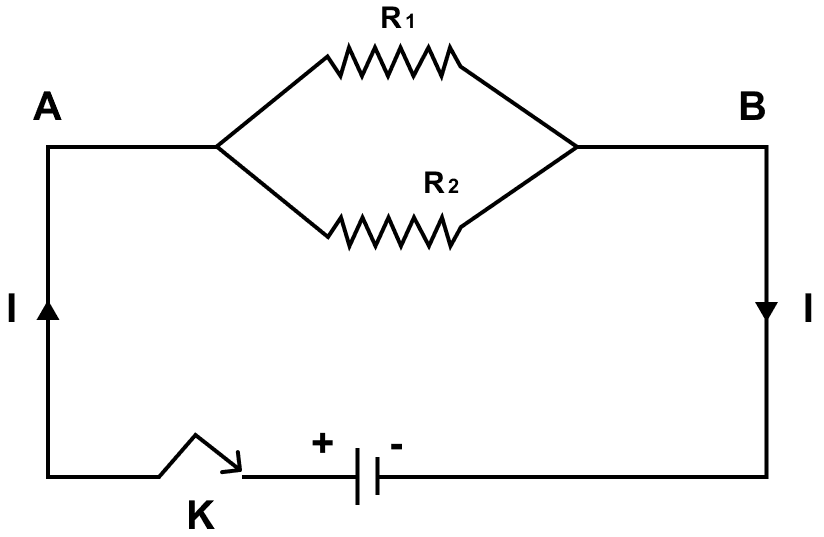Let I1 and I2 be the currents through the resistances R1 and R2 respectively, then total current drawn from the battery is

I = I1 + I2    [Equation 1]

If potential difference between the two ends A and B is V, then by Ohm's law

current in R1 is I1 = $\dfrac{V}{R_1}$

current in R2 is I2 = $\dfrac{V}{R_2}$

I1 + I2 = $\dfrac{V}{R_1}$ + $\dfrac{V}{R_2}$    [Equation 2]

If the equivalent resistance of the combination between the points A and C is Rp, then total current drawn from the source is

I = $\dfrac{V}{R_p}$    [Equation 3]

Substituting the values of I and I1 + I2 from equation 3 and 2 in 1, we get,

$\dfrac{V}{R_p} = V\Big(\dfrac{1}{R_1} + \dfrac{1}{R_2}\Big) \\[0.5em] \Rightarrow \dfrac{1}{R_p} = \dfrac{1}{R_1} + \dfrac{1}{R_2}$

Thus, in the parallel combination, the reciprocal of the equivalent resistance is equal to the sum of the reciprocals of the individual resistances.

#### Question 10

State how are the two resistors joined with a battery in each of the following cases when —

(a) same current flows in each resistor,

(b) potential difference is same across each resistor,

(c) equivalent resistance is less than either of the two resistances,

(d) equivalent resistance is more than either of the two resistances.

(a) When same current flows in each resistor, then the two resistors are joined in series

(b) When potential difference is same across each resistor, then the two resistors are joined in parallel

(c) When equivalent resistance is less than either of the two resistances, then the two resistors are joined in parallel

(d) When equivalent resistance is more than either of the two resistances, then the two resistors are joined in series

#### Question 11

The V-I graph for a series combination and for a parallel combination of two resistors is shown in figure. Which of the two, A or B, represents the parallel combination? Give a reason for your answer.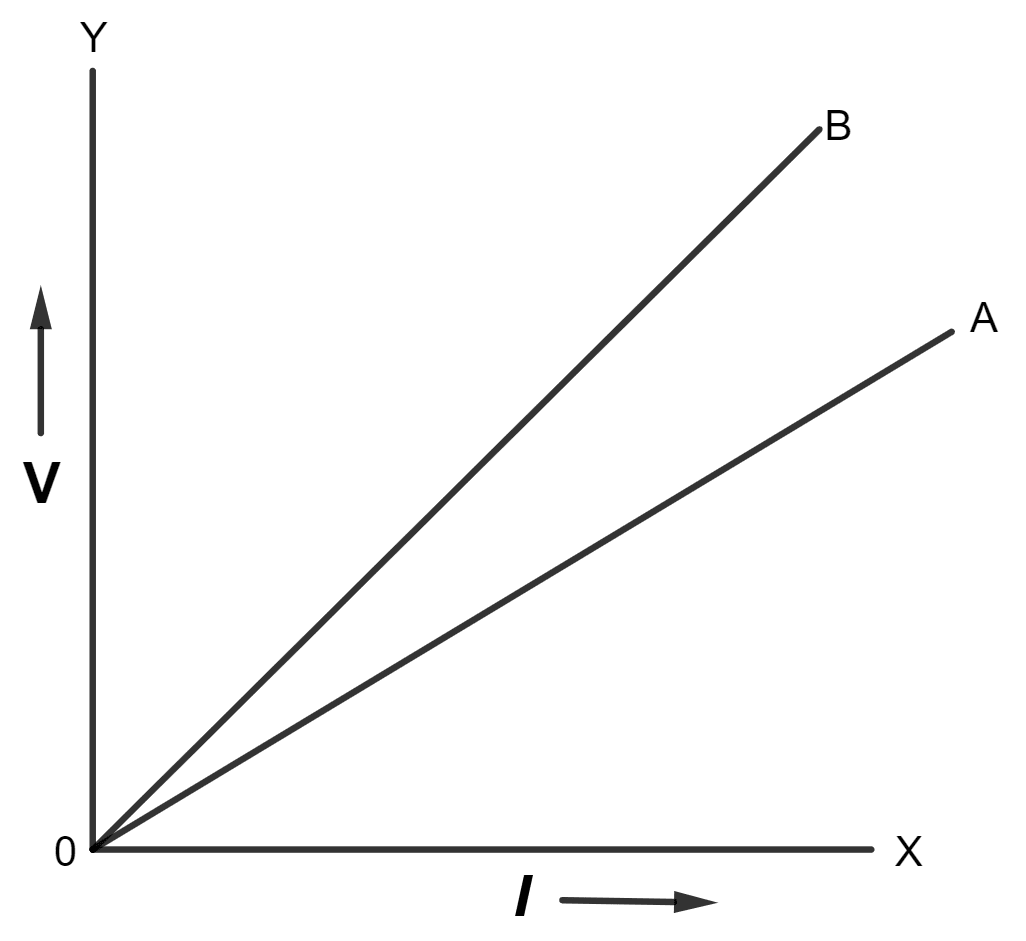The slope of V-I graph gives the resistance. Since the straight line A is less steeper than B, so the straight line A represents small resistance. In parallel combination, the equivalent resistance is less than in series combination so A represents the parallel combination.

## Multiple Choice Type

#### Question 1

In series combination of resistances —

1. p.d. is same across each resistance
2. total resistance is reduced
3. current is same in each resistance ✓
4. all of the above are true

Current is same in each resistance in series combination of resistances. The current has a single path to flow, hence, same current passes through each resistor.

#### Question 2

In parallel combination of resistances —

1. p.d. is same across each resistance ✓
2. total resistance is increased
3. current is same in each resistance
4. all of the above are true

Potential difference is same across each resistor in parallel combination of resistances, which is equal to the potential difference across the terminals of the battery (or source).

#### Question 3

Which of the following combinations have the same equivalent resistance between X and Y?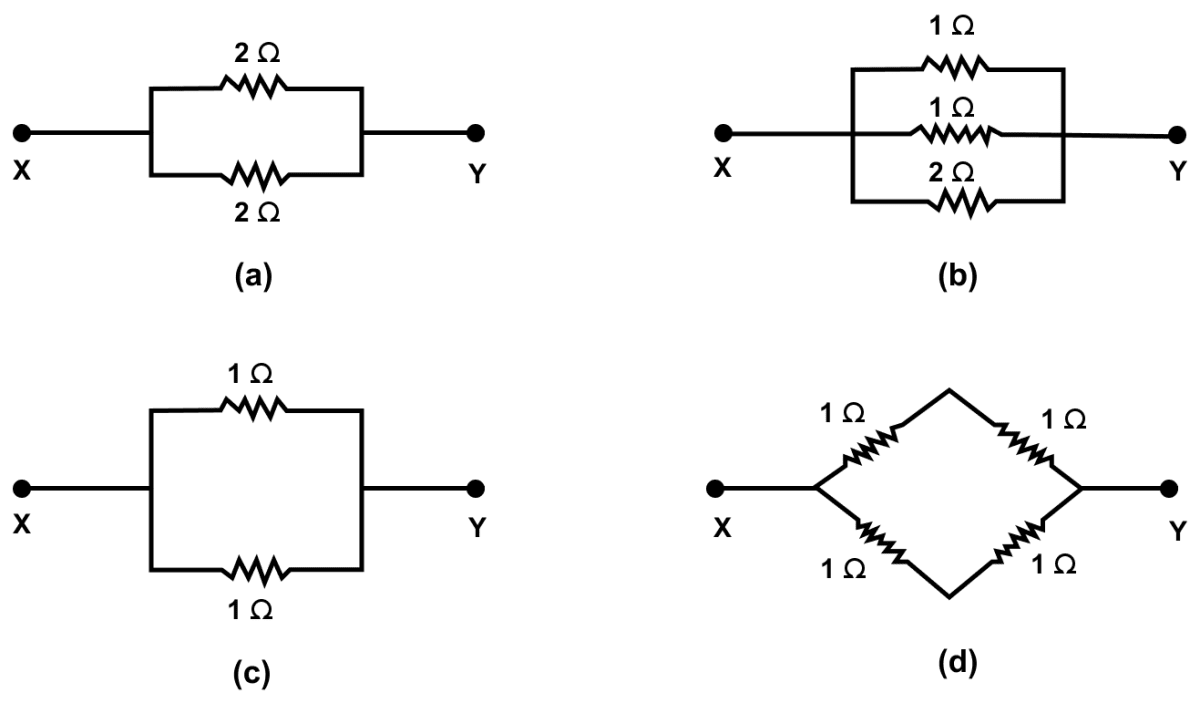(a) and (d) have the same equivalent resistance between X and Y.

(a) In the circuit, two resistors of 2 Ω each are connected in parallel. In parallel, the equivalent resistance is RP, then

$\dfrac{1}{R_P} = \dfrac{1}{2} + \dfrac{1}{2} \\[0.5em] \dfrac{1}{R_P} = \dfrac{2}{2} \\[0.5em] \Rightarrow R_P = 1 Ω$

Hence, RP = 1 Ω

(b) In the circuit, three resistors of 1 Ω, 1 Ω and 2 Ω are connected in parallel. In parallel, the equivalent resistance is RP, then

$\dfrac{1}{R_P} = \dfrac{1}{1} + \dfrac{1}{1} + \dfrac{1}{2} \\[0.5em] \dfrac{1}{R_P} = \dfrac{2 + 2 + 1}{2} \\[0.5em] \Rightarrow R_P = \dfrac{2}{5} Ω \\[0.5em] \Rightarrow R_P = 0.4 Ω$

Hence, RP = 0.4 Ω

(c) In the circuit two resistors of 1 Ω each are connected in parallel. In parallel, the equivalent resistance is RP, then

$\dfrac{1}{R_P} = \dfrac{1}{1} + \dfrac{1}{1} \\[0.5em] \dfrac{1}{R_P} = \dfrac{1 + 1}{1} \\[0.5em] \dfrac{1}{R_P} = \dfrac{2}{1} \\[0.5em] R_P = \dfrac{1}{2} \\[0.5em] \Rightarrow R_P = 0.5 Ω$

Hence, RP = 0.5 Ω

(d) In the circuit there are three parts. In the first part two resistor of 1 Ω each are connected in series. In series, the equivalent resistance is R'S, then

R'S = (1 + 1) Ω = 2 Ω

In the second part two resistor of 1 Ω each are connected in series. In series, the equivalent resistance is R''S, then

R''S = (1 + 1) Ω = 2 Ω

In the third part,R'S and R''S are in parallel, the equivalent resistance is RP, then

$\dfrac{1}{R_P} = \dfrac{1}{2} + \dfrac{1}{2} \\[0.5em] \dfrac{1}{R_P} = \dfrac{1 + 1}{2} \\[0.5em] \dfrac{1}{R_P} = \dfrac{2}{2} \\[0.5em] \Rightarrow R_P = 1 Ω$

Hence, RP = 1 Ω

Therefore, on observing the values we can say that (a) and (d) have same equivalent resistances i.e., 1 Ω.

## Numericals

#### Question 1

The diagram in figure shows a cell of e.m.f. ε = 2 volt and internal resistance r = 1 ohm connected to an external resistance R = 4 ohm. The ammeter A measures the current in the circuit and the voltmeter V measures the terminal voltage across the cell. What will be the readings of the ammeter and voltmeter when (i) the key K is open, and (ii) the key K is closed.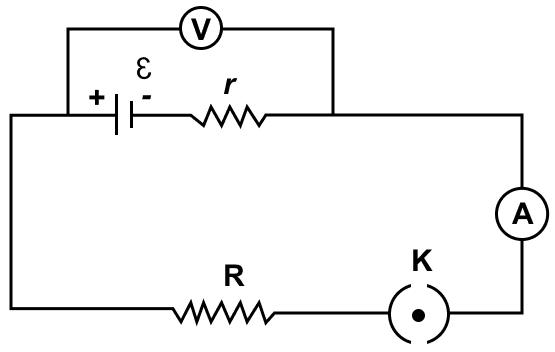Given,

e.m.f. ε = 2 volt

resistance r = 1 ohm

external resistance R = 4 ohm

(i) When the key is open then no current is flowing in the circuit and hence the ammeter reading = 0

From relation,

Voltage (V) = ε – Ir

Substituting the values in the formula we get,

V = 2 – (0 × 1)

V = 2 volt

Hence, voltmeter reading = 2 volt

(ii) When key is closed, current drawn from the cell,

$\text{I} = \dfrac{\text{e.m.f. of cell}}{\text{total resistance}} = \dfrac{\text{ε}}{\text{R} + \text{r}}$

Substituting the values in the formula above we get,

$I = \dfrac{2}{4 + 1} \\[0.5em] I = \dfrac{2}{5} \\[0.5em] \Rightarrow I = 0.4 \text{ ampere}$

Hence, ammeter reading = 0.4 ampere

From relation,

Voltage (V) = ε – Ir

Substituting the values in the formula we get,

V = 2 - (0.4 x 1) = 1.6 V

Hence, voltmeter reading = 1.6 volt

#### Question 2

A battery of e.m.f. 3.0 V supplies current through a circuit in which resistance can be changed. A high resistance voltmeter is connected across the battery. When the current is 1.5 A, the voltmeter reads 2.7 V. Find the internal resistance of the battery.

Given,

e.m.f. (ε) = 3.0 V

Current (I) = 1.5 A

Potential difference (V) = 2.7 V

r = ?

From relation,

V = ε – Ir

Substituting the values in the formula above we get,

$2.7 = 3 - (1.5 r) \\[0.5em] 1.5 r = 3 - 2.7 \\[0.5em] 1.5 r = 0.3 \\[0.5em] r = \dfrac{0.3}{1.5} \\[0.5em] r = \dfrac{3}{15} \\[0.5em] \Rightarrow r = 0.2 Ω$

Hence, internal resistance of the battery = 0.2 Ω

#### Question 3

A cell of emf 1.8 V and internal resistance 2 Ω is connected in series with an ammeter of resistance 0.7 Ω and resistance of 4.5 Ω as shown in figure.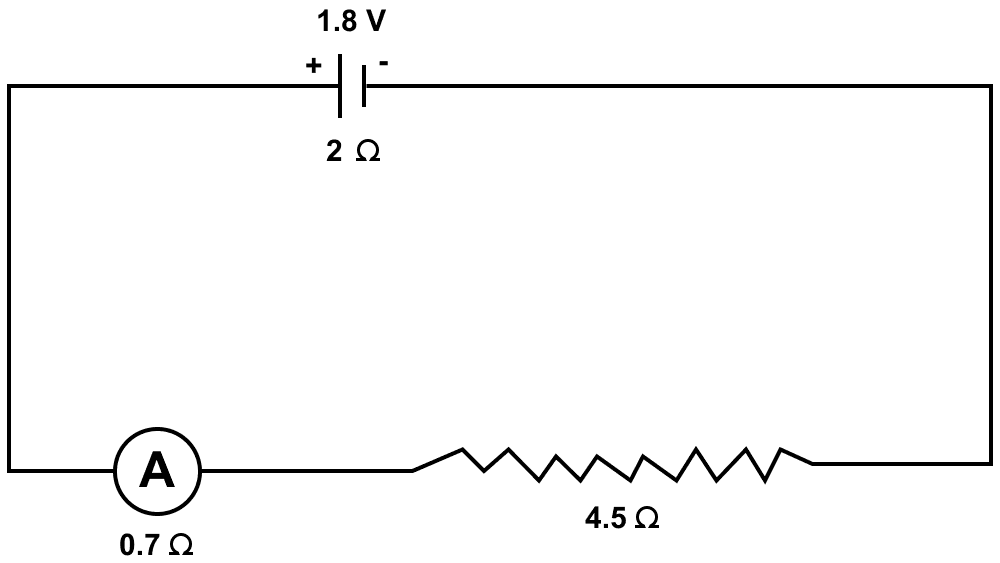(a) What would be the reading of the ammeter?

(b) What is the potential difference across the terminals of the cell ?

(a) Given,

e.m.f. (ε) = 1.8 V

Internal resistance (r) = 2 Ω

I = ?

Total resistance of arrangement = 2 + 0.7 + 4.5 = 7.2 Ω

From relation,

$I = \dfrac{ε}{R}$

Substituting the values in the formula above we get,

$I = \dfrac{1.8}{7.2} \\[0.5em] I = 0.25 A \\[0.5em]$

Hence, reading of ammeter = 0.25 A

(b) Current (I) = 0.25 A

total resistance (excluding internal resistance) = 4.5 + 0.7 = 5.2 ohm

Using ohm's law

V = IR

Substituting the values in the formula above we get,

V = 0.25 × 5.2

V = 1.3 V

Hence, potential difference across the terminals of the battery = 1.3 V

#### Question 4

A music system draws a current of 400 mA when connected to a 12 V battery.

(a) What is the resistance of the music system ?

(b) The music system if left playing for several hours and finally the battery voltage drops and the music system stops playing when the current drops to 320 mA. At what battery voltage does the music system stop playing.

Given,

I = 400 mA = 400 x 10-3 = 0.4 A

V = 12 V

From Ohm's law,

V = IR

Substituting the values in the formula we get,

$12 = 0.4 \times R \\[0.5em] \Rightarrow R = \dfrac{12}{0.4} \\[0.5em] \Rightarrow R = 30 Ω$

Hence, the resistance of the music system = 30 Ω

(b) Given,

I = 320 mA = 320 x 10-3 A = 0.32 A

R = 30 Ω

From Ohm's law,

V = IR

Substituting the values in the formula we get,

V = 0.32 x 30 = 9.6V

Hence, the battery voltage when the music system stops playing = 9.6 V

#### Question 5

A cell of e.m.f. ε and internal resistance r sends a current of 1.0 A when it is connected to an external resistance of 1.9 ohm. But it sends a current of 0.5 A when it is connected to an external resistance of 3.9 ohm. Calculate the values of ε and r.

Given,

e.m.f. = ε

internal resistance = r

current (I) = 1.0 A

external resistance (R) = 1.9 ohm

Case 1

From relation,

ε = I (R + r)

Substituting the value in the formula above we get,

ε = 1 (1.9 + r)

ε = 1.9 + r    [Equation 1]

In second case,

I = 0.5 A,

R = 3.9 Ω

Substituting the value in the formula above we get,

ε = 0.5 (3.9 + r)

ε = 1.95 + 0.5r    [Equation 2]

Equating 1 and 2 we get,

$1.9 + r = 1.95 + 0.5r \\[0.5em] r - 0.5 r = 1.95 - 1.9 \\[0.5em] 0.5 r = 0.05 \\[0.5em] \Rightarrow r = \dfrac{0.05}{0.5} \\[0.5em] \Rightarrow r = 0.1 Ω$

Now, substituting the value of r in equation 1, we get,

ε = 1.9 + 0.1

ε = 2 V

Hence, ε = 2 V , r = 0.1 Ω

#### Question 6

Two resistors having resistance 4 Ω and 6 Ω are connected in parallel. Find their equivalent resistance.

In parallel, if the equivalent resistance is RP, then

$\dfrac{1}{R_P} = \dfrac{1}{R_1} + \dfrac{1}{R_2} \\[0.5em] \dfrac{1}{R_P} = \dfrac{1}{4} + \dfrac{1}{6} \\[0.5em] \dfrac{1}{R_P} = \dfrac{3 +2}{12} \\[0.5em] \dfrac{1}{R_P} = \dfrac{5}{12} \\[0.5em] R_P = \dfrac{12}{5} \\[0.5em] \Rightarrow R_P = 2.4 Ω$

Hence, equivalent resistance = 2.4 Ω

#### Question 7

Four resistors each of resistance 2 ohm are connected in parallel. What is the effective resistance?

Give,

R1 = R2 = R3 = R4 = 2 Ω

In parallel, if the equivalent resistance is RP, then

$\dfrac{1}{R_P} = \dfrac{1}{R_1} + \dfrac{1}{R_2} + \dfrac{1}{R_3} + \dfrac{1}{R_4} \\[0.5em] \dfrac{1}{R_P} = \dfrac{1}{2} + \dfrac{1}{2} + \dfrac{1}{2} + \dfrac{1}{2} \\[0.5em] \dfrac{1}{R_P} = \dfrac{4}{2} \\[0.5em] \dfrac{1}{R_P} = 2 \\[0.5em] \Rightarrow R_P = 0.5 Ω$

Hence, equivalent resistance = 0.5 Ω

#### Question 8

You have three resistors of values 2 Ω, 3 Ω and 5 Ω . How will you join them so that the total resistance is less than 1 Ω ? Draw diagram and find the total resistance.

In order to get a total resistance less than 1 Ω, the three resistors of values 2 Ω, 3 Ω and 5 Ω, should be connected in parallel as shown in the diagram below: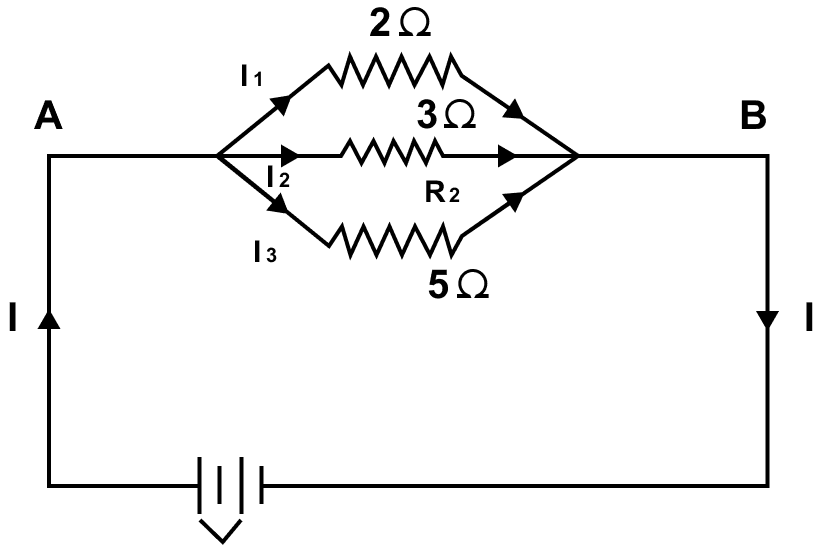In parallel, if the equivalent resistance is RP, then

$\dfrac{1}{R_P} = \dfrac{1}{R_1} + \dfrac{1}{R_2} + \dfrac{1}{R_3} \\[0.5em] \dfrac{1}{R_P} = \dfrac{1}{2} + \dfrac{1}{3} + \dfrac{1}{5} \\[0.5em] \dfrac{1}{R_P} = \dfrac{15 + 10+ 6}{30} \\[0.5em] \dfrac{1}{R_P} = \dfrac{31}{30} \\[0.5em] \Rightarrow R_P = 0.97 Ω$

Hence, the three resistors should be connected in parallel and total resistance = 0.97 Ω

#### Question 9

Three resistors each of 2 Ω are connected together so that their total resistance is 3 Ω. Draw a diagram to show this arrangement and check it by calculation.

When two resistors (2 Ω each) in parallel combination are connected to the third resistor (2 Ω) in series connection then the resultant resistance is 3 Ω. The below diagram shows this arrangement of resistors: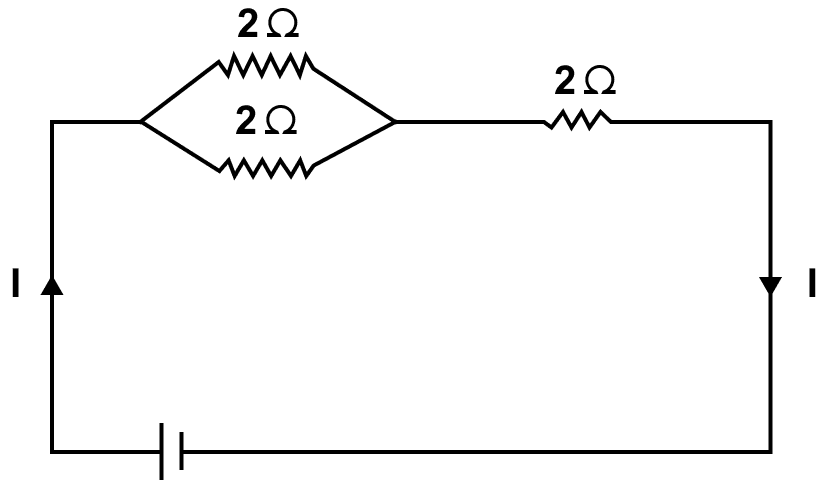To verify,

Total resistance of two resistors in parallel,

In parallel, if the equivalent resistance is RP, then

$\dfrac{1}{R_P} = \dfrac{1}{R_1} + \dfrac{1}{R_2} \\[0.5em] \dfrac{1}{R_P} = \dfrac{1}{2} + \dfrac{1}{2} \\[0.5em] \dfrac{1}{R_P} = \dfrac{2}{2} \\[0.5em] \Rightarrow R_P = 1 Ω$

Hence, RP = 1 Ω

In series connection,

Equivalent resistance = RE = RP + R3
= 1 + 2 = 3 Ω

Hence, Equivalent resistance = 3 Ω

#### Question 10

Calculate the equivalent resistance between the points A and B in figure if each resistance is 2.0 Ω.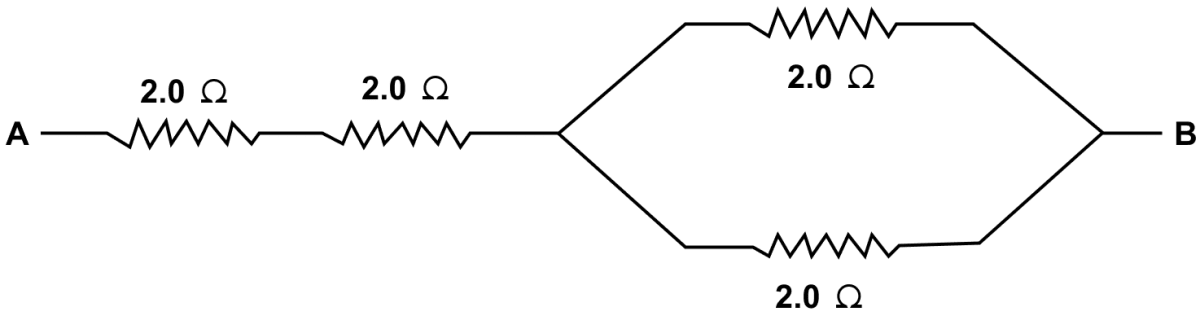Let the resistors be R1, R2, R3, R4 each of 2.0 Ω.

In parallel, if the equivalent resistance is RP, then

$\dfrac{1}{R_P} = \dfrac{1}{R_3} + \dfrac{1}{R_4} \\[0.5em] \dfrac{1}{R_P} = \dfrac{1}{2} + \dfrac{1}{2} \\[0.5em] \dfrac{1}{R_P} = \dfrac{2}{2} \\[0.5em] \Rightarrow R_P = 1 Ω$

Hence, RP = 1 Ω

In series connection,

Equivalent resistance = RE = R1 + R2 + RP
= 2 + 2 + 1 = 5 Ω

Hence, Equivalent resistance = 5 Ω

#### Question 11

A combination consists of three resistors in series. Four similar sets are connected in parallel. If the resistance of each resistor is 2 ohm, find the resistance of the combination.

Let the four set of resistors be R1, R2, R3, R4 .

As each set consists of three resistors of 2 Ω each in series:
Resistance of each set = 2 + 2 + 2 = 6 Ω

As four similar sets of equivalent resistance 6 Ω are arranged in parallel, so the equivalent resistance (RP) will be:

$\dfrac{1}{R_P} = \dfrac{1}{R_1} + \dfrac{1}{R_2} + \dfrac{1}{R_3} + \dfrac{1}{R_4} \\[0.5em] \dfrac{1}{R_P} = \dfrac{1}{6} + \dfrac{1}{6} + \dfrac{1}{6} + \dfrac{1}{6} \\[0.5em] \dfrac{1}{R_P} = \dfrac{4}{6} \\[0.5em] R_P = \dfrac{6}{4} \\[0.5em] \Rightarrow R_P = 1.5 Ω$

Hence, equivalent resistance = 1.5 Ω

#### Question 12

In the circuit shown below in figure, calculate the value of x if the equivalent resistance between the points A and B is 4 Ω.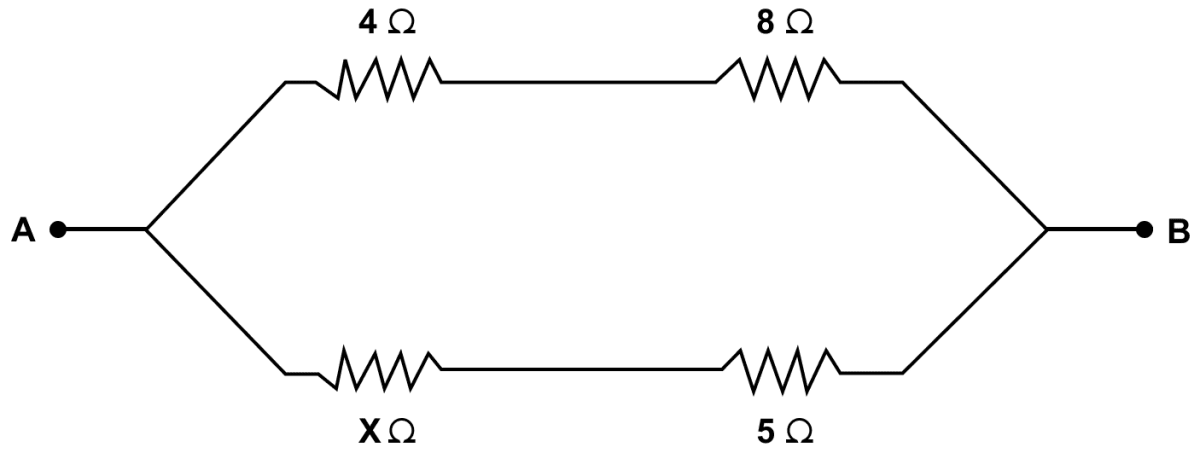In the circuit, there are three parts. In the first part, resistors of 4 Ω and 8 Ω are connected in series. If the equivalent resistance of this part is R's then

R's = 4 + 8 = 12 Ω

In the second part, resistors of x Ω and 5 Ω are connected in series. If the equivalent resistance of this part is R''s then

R''s = (x + 5) Ω

In the third part, the two parts of resistance R's = 12 Ω and R''s = (x + 5) Ω are connected in parallel. If the equivalent resistance between points A and B is Rp then

$\dfrac{1}{R_p} = \dfrac{1}{R'_s} + \dfrac{1}{R''_s} \\[0.5em] \dfrac{1}{R_p} = \dfrac{1}{12} + \dfrac{1}{x + 5} \\[0.5em]$

But it is given that equivalent resistance between points A and B is 4 Ω
∴ Rp = 4 Ω

Putting value of Rp in the equation above and solving for x:

$\dfrac{1}{4} = \dfrac{1}{12} + \dfrac{1}{x + 5} \\[0.5em] \dfrac{1}{4} = \dfrac{x + 5 +12}{12(x+5)} \\[0.5em] \dfrac{1}{4} = \dfrac{x + 17}{12(x+5)} \\[0.5em] \dfrac{12(x+5)}{4} = x + 17 \\[0.5em] 3(x+5) = x + 17 \\[0.5em] 3x + 15 = x + 17 \\[0.5em] 3x - x = 17 - 15 \\[0.5em] 2x = 2 \\[0.5em] \Rightarrow x = 1 Ω$

Hence, the value of x = 1 Ω

#### Question 13

Calculate the effective resistance between the points A and B in the circuit shown in figure.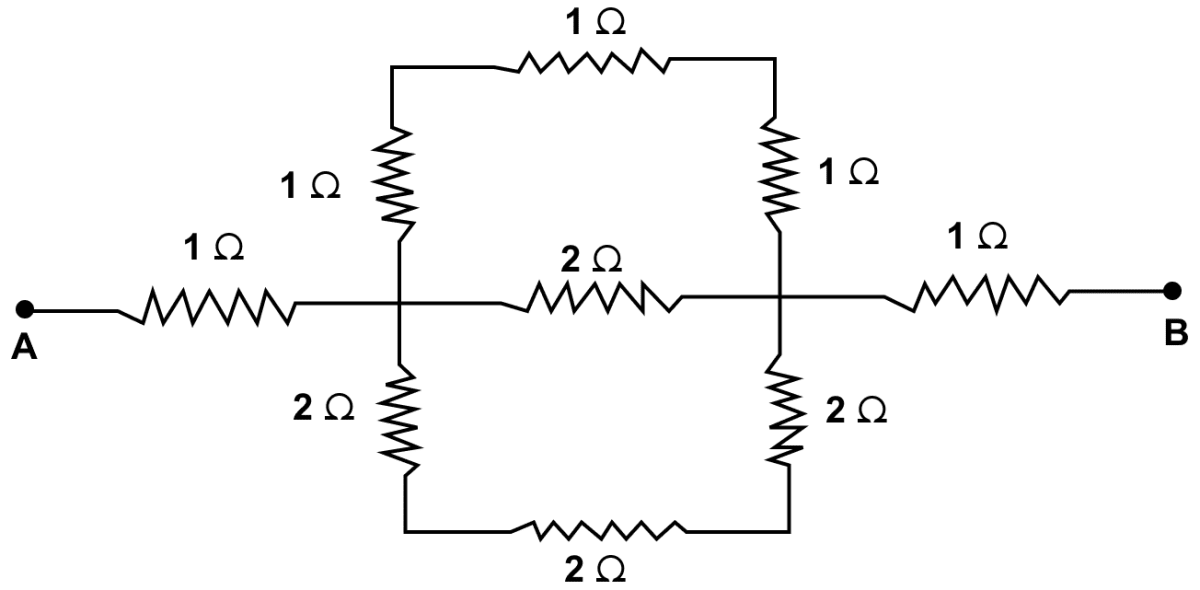In the circuit, there are four parts. In the first part, three resistors of 1 Ω each are connected in series. If the equivalent resistance of this part is R's then

R's = (1 + 1 + 1) Ω = 3 Ω

In the second part, three resistors of 2 Ω each are connected in series. If the equivalent resistance of this part is R''s then

R''s = (2 + 2 + 2) Ω = 6 Ω

In the third part, the two parts of resistance R's = 3 Ω and R''s = 6 Ω and 2 Ω are connected in parallel. If the equivalent resistance is Rp then

$\dfrac{1}{R_p} = \dfrac{1}{R'_s} + \dfrac{1}{2} + \dfrac{1}{R''_s} \\[0.5em] \dfrac{1}{R_p} = \dfrac{1}{3} + \dfrac{1}{2} + \dfrac{1}{6} \\[0.5em] \dfrac{1}{R_p} = \dfrac{2 + 3 + 1}{6} \\[0.5em] \dfrac{1}{R_p} = \dfrac{6}{6} \\[0.5em] \Rightarrow R_p = 1 Ω \\[0.5em]$

∴ Rp = 1 Ω

In the fourth part 1 Ω, (Rp = 1 Ω) and 1 Ω are connected in series in between points A and B.

Hence, the equivalent resistance is 1 + 1 + 1 = 3 Ω

#### Question 14

A uniform wire with a resistance of 27 ohm is divided into three equal pieces and then they are joined in parallel. Find the equivalent resistance of the parallel combination.

Given, uniform wire with a resistance of 27 ohm is divided into three equal pieces. Hence, resistance of each piece = 9 Ω.

Three such pieces are joined in parallel.

In parallel, if the equivalent resistance is RP, then

$\dfrac{1}{R_P} = \dfrac{1}{R_1} + \dfrac{1}{R_2} + \dfrac{1}{R_3} \\[0.5em] \dfrac{1}{R_P} = \dfrac{1}{9} + \dfrac{1}{9} + \dfrac{1}{9} = \dfrac{3}{9} = \dfrac{1}{3} \\[0.5em] \Rightarrow R_P = 3 Ω$

Hence, equivalent resistance = 3 Ω

#### Question 15

A circuit consists of a resistor of 1 ohm in series with a parallel arrangement of resistors of 6 ohm and 3 ohm. Calculate the total resistance of the circuit. Draw a diagram of the arrangement.

Below diagram shows the arrangement of resistors: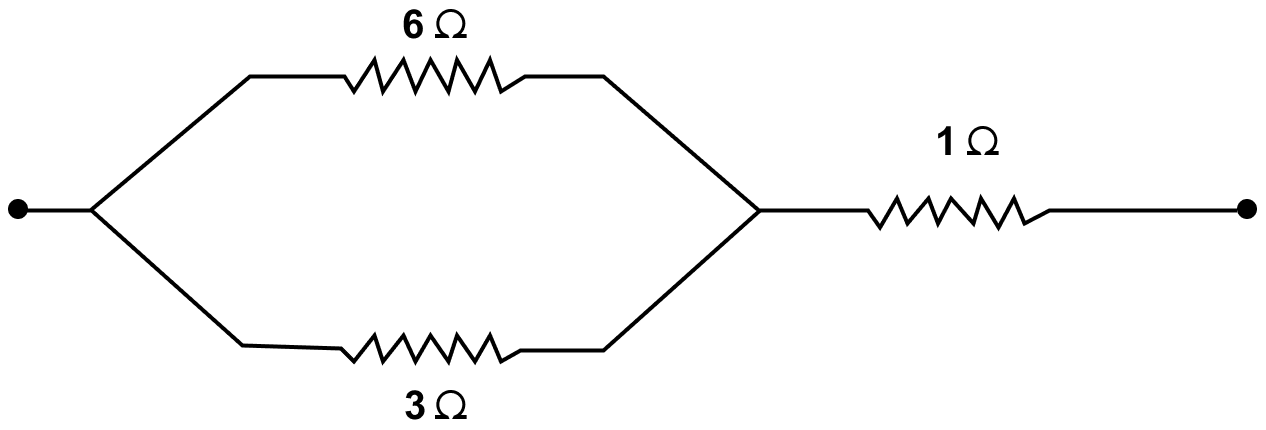In the circuit, there are two parts. In the first part, two resistors of 6 Ω and 3 Ω are connected in parallel. If the equivalent resistance is Rp then

$\dfrac{1}{R_P} = \dfrac{1}{6} + \dfrac{1}{3} \\[0.5em] \dfrac{1}{R_P} = \dfrac{1+2}{6} = \dfrac{3}{6} = \dfrac{1}{2} \\[0.5em] \Rightarrow R_P = 2 Ω$

Hence, RP = 2 Ω

In the second part, 1 Ω and (RP = 2 Ω) are connected in series. If the equivalent resistance is Rs then

Rs = 1 + RP = 1 + 2 = 3 Ω

Hence, total resistance of the circuit = 3 Ω

#### Question 16

Calculate the effective resistance between the points A and B in the network shown below in figure.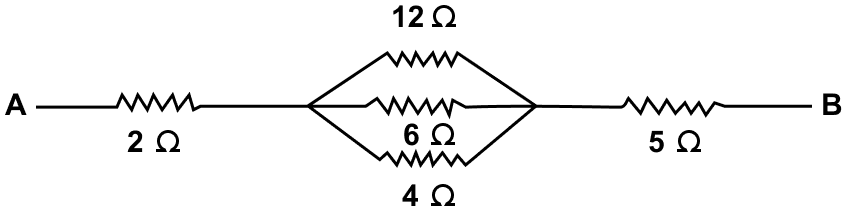In the circuit, there are two parts. In the first part, resistors of 12 Ω, 6 Ω and 4 Ω are connected in parallel. If the equivalent resistance of this part is R'p then

$\dfrac{1}{{R'}_P} = \dfrac{1}{12} + \dfrac{1}{6} + \dfrac{1}{4} \\[0.5em] \dfrac{1}{{R'}_P} = \dfrac{1 + 2 + 3}{12} \\[0.5em] \dfrac{1}{{R'}_P} = \dfrac{6}{12} \\[0.5em] \dfrac{1}{{R'}_P} = \dfrac{1}{2} \\[0.5em] \Rightarrow {R'}_P = 2 Ω$

Hence, R'P = 2 Ω

In the second part, resistors of 2 Ω, (R'p = 2Ω) and 5 Ω are connected in series. If the equivalent resistance of this part is Rs then

Rs = (2 + 2 + 5) Ω = 9 Ω

Hence, Effective resistance between A and B = 9 Ω

#### Question 17

Calculate the equivalent resistance between the points A and B in figure.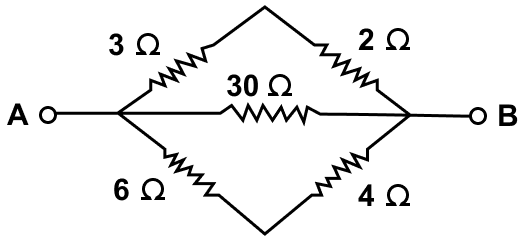In the circuit, there are three parts. In the first part, two resistors of 3 Ω, 2 Ω are connected in series. If the equivalent resistance is R's then

R's = 3 + 2 = 5 Ω

In the second part, two resistors of 6 Ω, 4 Ω are connected in series. If the equivalent resistance is R''s then

R''s = 6 + 4 = 10 Ω

In the third part R's, 30 Ω and R''s are connected in parallel. If the equivalent resistance is Rp then

$\dfrac{1}{R_P} = \dfrac{1}{R'_s} + \dfrac{1}{30} + \dfrac{1}{R''_s} \\[0.5em] \dfrac{1}{R_P} = \dfrac{1}{5} + \dfrac{1}{30} + \dfrac{1}{10} \\[0.5em] \dfrac{1}{R_P} = \dfrac{6 + 1 + 3}{30} \\[0.5em] \dfrac{1}{R_P} = \dfrac{10}{30} \\[0.5em] \dfrac{1}{R_P} = \dfrac{1}{3} \\[0.5em] \Rightarrow R_P = 3 Ω$

Hence, RP = 3 Ω

#### Question 18

In the network shown in figure, calculate the equivalent resistance between the points (a) A and B (b) A and C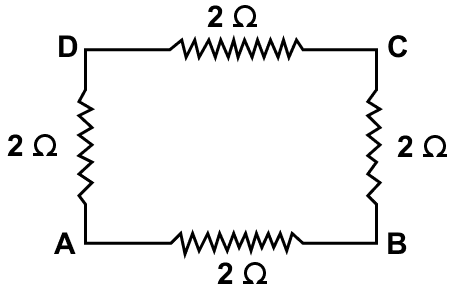In the circuit, there are two parts. In the first part, three resistors of 2 Ω each are connected in series. If the equivalent resistance of this part is R's then

R's = (2 + 2 + 2) Ω = 6 Ω

In the second part, the resistance of first part (R's = 6 Ω ) and 2 Ω are connected in parallel. If the equivalent resistance of this part is Rp then

$\dfrac{1}{R_p} = \dfrac{1}{6} + \dfrac{1}{2} \\[0.5em] \dfrac{1}{R_p} = \dfrac{1 + 3}{6} \\[0.5em] \dfrac{1}{R_p} = \dfrac{4}{6} \\[0.5em] \Rightarrow R_p = 1.5 Ω \\[0.5em]$

∴ Rp = 1.5 Ω

(b) In the circuit, there are three parts. In the first part, two resistors of 2 Ω each are connected in series. If the equivalent resistance of this part is R's then

R's = (2 + 2) Ω = 4 Ω

In the second part, two resistors of 2 Ω each are connected in series. If the equivalent resistance of this part is R''s then

R''s = (2 + 2) Ω = 4 Ω

In the third part, the two parts of resistance R's = 4 Ω and R''s = 4 Ω are connected in parallel. If the equivalent resistance between points A and C is Rp then

$\dfrac{1}{R_p} = \dfrac{1}{4} + \dfrac{1}{4} \\[0.5em] \dfrac{1}{R_p} = \dfrac{2}{4} \\[0.5em] \dfrac{1}{R_p} = \dfrac{1}{2} \\[0.5em] \Rightarrow R_p = 2 Ω \\[0.5em]$

∴ Equivalent Resistance between A and C = 2 Ω

#### Question 19

Five resistors, each of 3 ohm, are connected as shown in figure. Calculate the resistance (a) between the points P and Q, and (b) between the points X and Y.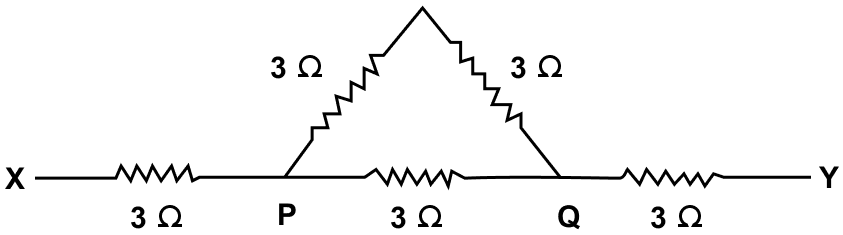(a) In the circuit, there are two parts. In the first part, two resistors of 3 Ω each are connected in series. If the equivalent resistance of this part is R's then

R's = (3 + 3) Ω = 6 Ω

In the second part, resistance R's = 6 Ω and 3 Ω are connected in parallel. If the equivalent resistance between points P and Q is Rp then

$\dfrac{1}{R_p} = \dfrac{1}{6} + \dfrac{1}{3} \\[0.5em] \dfrac{1}{R_p} = \dfrac{1 + 2}{6} \\[0.5em] \dfrac{1}{R_p} = \dfrac{3}{6} \\[0.5em] \Rightarrow R_p = 2 Ω \\[0.5em]$

Equivalent resistance between the points P and Q = 2 Ω

(b) In the circuit, 3 Ω, Rp = 2 Ω and 3 Ω are connected in series. If the equivalent resistance of this part is Rs then

Rs = (3 + 2 + 3) Ω = 8 Ω

Equivalent resistance between the points X and Y = 8 Ω

#### Question 20

Two resistors of 2 Ω and 3 Ω are connected (a) in series, (b) in parallel, with a battery of 6.0 V and negligible internal resistance. For each case draw a circuit diagram and calculate the current through the battery.

(a) Circuit diagram showing two resistors of 2 Ω and 3 Ω are connected in series with a battery of 6.0 V and negligible internal resistance is shown below: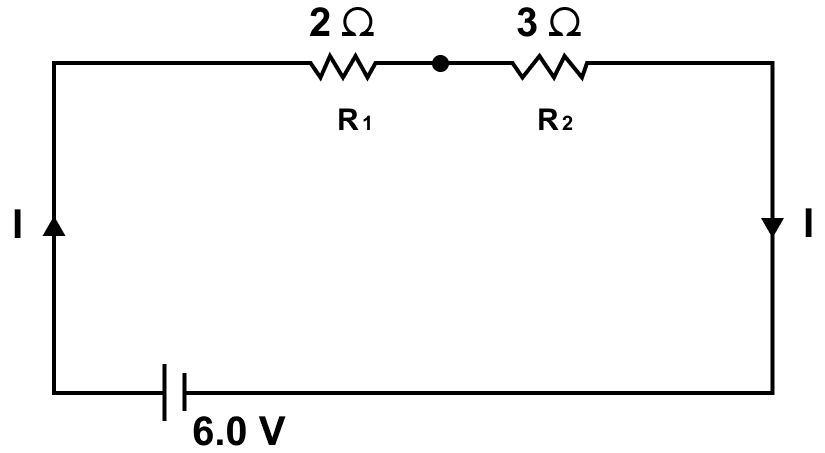Given,

Two resistors of 2 Ω and 3 Ω are connected in series. If the equivalent resistance of this part is R's then

R's = (2 + 3) Ω = 5 Ω

Potential Difference V = 6 V

Current I = ?

From Ohm's law

V = IR

Substituting the values in the formula above, we get,

6 = I x 5
⇒ I = 6 / 5 = 1.2 A

Hence, in series, current through the battery = 1.2 A

(b) Circuit diagram showing two resistors of 2 Ω and 3 Ω are connected in parallel with a battery of 6.0 V and negligible internal resistance is shown below: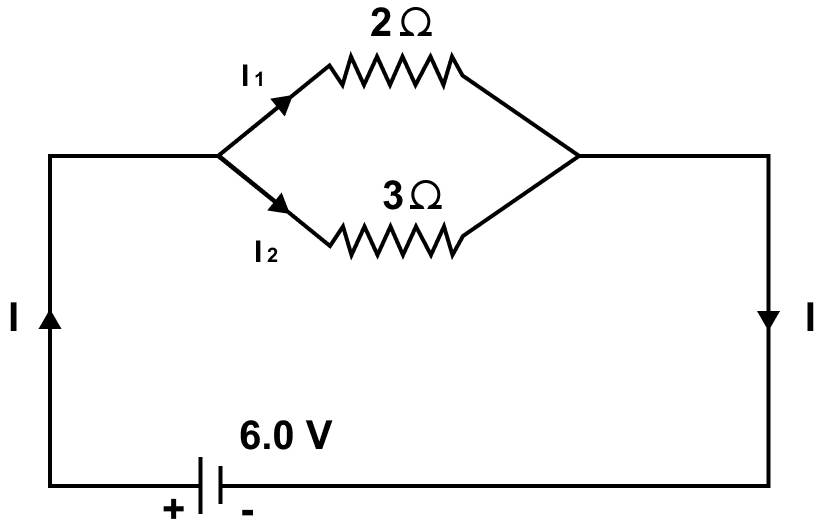Given,

Two resistors of 2 Ω and 3 Ω are connected in parallel. If the equivalent resistance of this part is Rp then

$\dfrac{1}{R_p} = \dfrac{1}{2} + \dfrac{1}{3} \\[0.5em] \dfrac{1}{R_p} = \dfrac{3 + 2}{6} = \dfrac{5}{6} \\[0.5em] R_p = \dfrac{6}{5} = 1.2 Ω$

Potential Difference V = 6 V

Current I = ?

From Ohm's law

V = IR

Substituting the values in the formula above, we get,

6 = I x 1.2
⇒ I = 6 / 1.2 = 5 A

Hence, in parallel, current through the battery = 5 A

#### Question 21

A resistor of 6 Ω is connected in series with another resistor of 4 Ω. A potential difference of 20 V is applied across the combination. Calculate (a) the current in the circuit and (b) potential difference across the 6 Ω resistor.

(a) Given,

Two resistors of 6 Ω and 4 Ω are connected in series. If the equivalent resistance of this part is R's then

R's = (6 + 4) Ω = 10 Ω

Potential Difference V = 20 V

Current I = ?

From Ohm's law

V = IR

Substituting the values in the formula above, we get,

20 = I x 10
⇒ I = 20 / 10 = 2 A

Hence, in series, current through the battery = 2 A

(b) Given,

Resistance R = 6 Ω

Potential Difference V = ?

Current I = 2 A

From Ohm's law

V = IR

Substituting the values in the formula above, we get,

V = 2 x 6 = 12 V

Hence, potential difference = 12 V

#### Question 22

Two resistors of resistance 4 Ω and 6 Ω are connected in parallel to a cell to draw 0.5 A current from the cell.

(a) Draw a labelled diagram of the arrangement.

(b) Calculate the current in each resistor.

(a) Labelled diagram of the arrangement is shown below: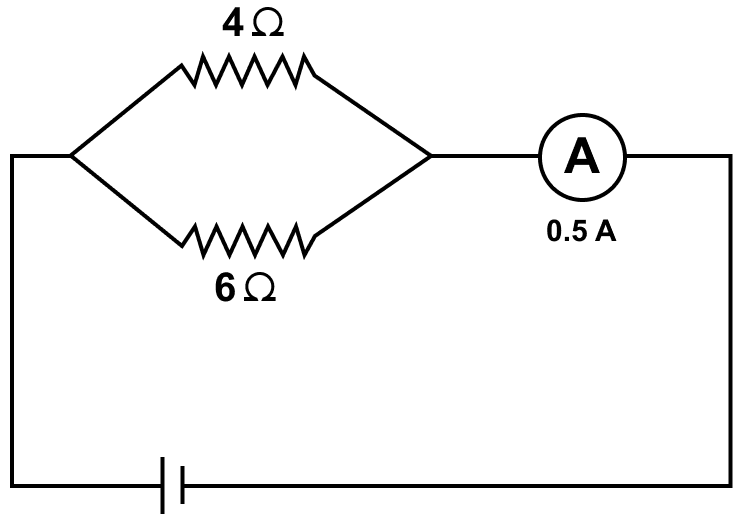(b) Two resistors of 4 Ω and 6 Ω are connected in parallel. If the equivalent resistance of this part is Rp then

$\dfrac{1}{R_p} = \dfrac{1}{4} + \dfrac{1}{6} \\[0.5em] \dfrac{1}{R_p} = \dfrac{3 + 2}{12} \\[0.5em] \dfrac{1}{R_p} = \dfrac{5}{12} \\[0.5em] R_p = \dfrac{12}{5} \\[0.5em] \Rightarrow R_p = 2.4 Ω$

Hence, equivalent resistance = 2.4 Ω

Current I = 0.5 A

Potential Difference V = ?

From Ohm's law

V = IR

Substituting the values in the formula above, we get,

V = 0.5 x 2.4 = 1.2 V

Current through 4 Ω = ?

From Ohm's law

V = IR

Substituting the values in the formula above, we get,

1.2 = I x 4
⇒ I = 1.2 / 4 = 0.3 A

Hence, current through 4 Ω resistor = 0.3 A

Current through 6 Ω = ?

From Ohm's law

V = IR

Substituting the values in the formula above, we get,

1.2 = I x 6
⇒ I = 1.2 / 6 = 0.2 A

Hence, current through 6 Ω resistor = 0.2 A

#### Question 23

Calculate the current flowing through each of the resistors A and B in the circuit shown in figure.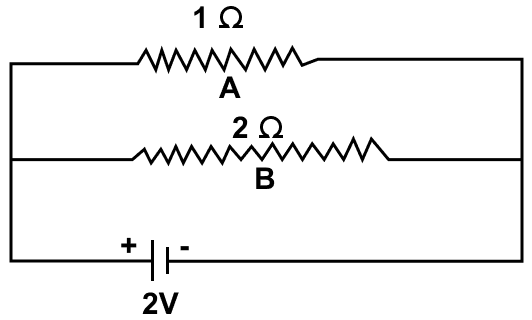(a) Given,

R = 1 Ω

V = 2 V

Current through 1 Ω = ?

From Ohm's law

V = IR

Substituting the values in the formula above, we get,

2 = I x 1
⇒ I = 2 / 1 = 2 A

Hence, current through Resistor A = 2 A

(b) Given,

R = 2 Ω

V = 2 V

Current through 2 Ω = ?

From Ohm's law

V = IR

Substituting the values in the formula above, we get,

2 = I x 2
⇒ I = 2 / 2 = 1 A

Hence, current through Resistor B = 1 A

#### Question 24

In figure, calculate —

(a) the total resistance of the circuit,

(b) the value of R, and

(c) the current flowing in R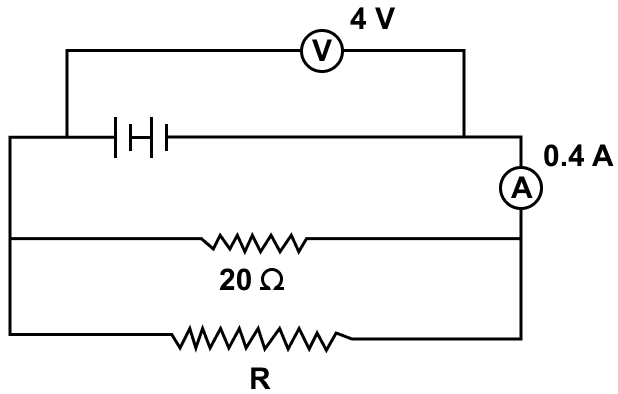(a) Given,

V = 4 V

I = 0.4 A

From Ohm's law

V = IR

4 = 0.4 x R
⇒ R = 4 / 0.4 = 10 Ω

Hence, total resistance of the circuit = 10 Ω

(b) As total resistance of the circuit is equal to 10 Ω and the two resistors R and 20 Ω are connected in parallel, hence we get,

$\dfrac{1}{10} = \dfrac{1}{R} + \dfrac{1}{20} \\[0.5em] \dfrac{1}{R} = \dfrac{1}{10} - \dfrac{1}{20} \\[0.5em] \dfrac{1}{R} = \dfrac{2 - 1}{20} \\[0.5em] \dfrac{1}{R} = \dfrac{1}{20} \\[0.5em] R = 20 Ω \\[0.5em]$

Hence, the value of R = 20 Ω

(c) current flowing in R = ?

V = 4 V

R = 20 Ω

I = ?

From Ohm's law

V = IR
⇒ 4 = I x 20
⇒ I = 4 / 20 = 1 / 5 = 0.2 A

Hence, the current flowing in R = 0.2 A

#### Question 25

A particular resistance wire has a resistance of 3.0 ohm per meter. Find —

(a) The total resistance of three lengths of this wire each 1.5 m long, joined in parallel.

(b) The potential difference of the battery which gives a current of 2.0 A in each of the 1.5 m length when connected in parallel to the battery (assume that resistance of the battery is negligible).

(c) The resistance of 5 m length of a wire of the same material, but with twice the area of cross section.

(a) Resistance of 1 m of wire = 3 Ω.

Resistance of 1.5 m of wire = 3 x 1.5 = 4.5 Ω. As three such wires are joined in parallel and if the equivalent resistance of this part is Rp then

$\dfrac{1}{R_p} = \dfrac{1}{4.5} + \dfrac{1}{4.5} + \dfrac{1}{4.5} \\[0.5em] \dfrac{1}{R_p} = \dfrac{3}{4.5} \\[0.5em] \Rightarrow R_p = \dfrac{4.5}{3} \\[0.5em] \Rightarrow R_p = 1.5 Ω$

Hence, total resistance of circuit = 1.5 Ω

(b) I = 2 A

From Ohm's Law

V = IR

Substituting the values in the formula above we get,

V = 2 x 4.5 = 9 V

Hence, potential difference = 9 V

(c) R = 3 Ω for 1 meter wire

Therefore, for 5 m

R = 3 x 5 = 15 Ω

Here the area is twice and resistance is inversely proportional to area.

Thus, resistance becomes half

R = 15 / 2 = 7.5 Ω

Hence, resistance = 7.5 Ω

#### Question 26

A cell supplies a current of 1.2 A through two resistors each of 2 Ω connected in parallel. When the resistors are connected in series, it supplies a current of 0.4 A. Calculate (i) the internal resistance and (ii) e.m.f. of the cell.

Given,

Two resistors each of 2 Ω connected in parallel. If the equivalent resistance of this part is Rp then

$\dfrac{1}{R_p} = \dfrac{1}{2} + \dfrac{1}{2} \\[0.5em] \dfrac{1}{R_p} = \dfrac{2}{2} \\[0.5em] \Rightarrow R_p = 1 Ω$

Hence, equivalent resistance Rp = 1 Ω

Given,

I = 1.2 A

From relation,

ε = I (R + r)

ε = 1.2 (1 + r)

ε = 1.2 + 1.2r    [Equation 1]

When the resistors are connected in series, it supplies a current of 0.4 A,

If the equivalent resistance of this part is Rs then

Rs = 2 + 2 = 4 Ω

Hence, equivalent resistance Rs = 4 Ω

Given, I = 0.4 A

From relation,

ε = I (R + r)

ε = 0.4 (4 + r)

ε = 1.6 + 0.4r    [Equation 2]

Equating 1 and 2, we get,

$1.2 + 1.2r = 1.6 + 0.4r \\[0.5em] 1.2r – 0.4r = 1.6 – 1.2 \\[0.5em] 0.8r = 0.4 \\[0.5em] r = \dfrac{0.4}{0.8} \\[0.5em] r = 0.5 Ω \\[0.5em]$

Hence, Internal resistance r = 0.5 Ω

(ii) Substituting the value in equation 1 we get,

ε = 1.2(1 + r)
= 1.2 (1 + 0.5)
= 1.2 x 1.5
= 1.8 V

Hence, e.m.f. of the cell = 1.8 V

#### Question 27

A battery of e.m.f. 15 V and internal resistance 3 Ω is connected to two resistors 3 Ω and 6 Ω connected in parallel. Find (a) the current through the battery (b) p.d. between the terminals of the battery (c) the current in 3 Ω resistor (d) the current in 6 Ω resistor.

(a) Given,

e.m.f. = 15 V

internal resistance r = 3 Ω

current through battery = ?

If Rp is the equivalent resistance of resistors 3 Ω and 6 Ω connected in parallel, then

$\dfrac{1}{R_p} = \dfrac{1}{3} + \dfrac{1}{6} \\[0.5em] \dfrac{1}{R_p} = \dfrac{2 + 1}{6} \\[0.5em] \dfrac{1}{R_p} = \dfrac{3}{6} \\[0.5em] \dfrac{1}{R_p} = \dfrac{1}{2} \\[0.5em] \Rightarrow R_p = 2 Ω$

From relation,

ε = I (R + r)

Substituting the value in the formula above we get,

15 = I(2 + 3)
⇒ 15 = I x 5
⇒ I = 15 / 5 = 3 A

Hence, current through the battery = 3 A

(b) Potential difference between the terminals of the battery = ?

Using Ohm's law

V = IR

R = 2 Ω

I = 3 A

Substituting the values in the formula above we get,

V = 3 x 2 = 6 V

Hence, potential difference between the terminals of the battery = 6 V

(c) Current in 3 Ω resistor = ?

Using Ohm's law

V = IR

R = 3 Ω

V = 6 V

I = ?

Substituting the values in the formula above we get,

$6 = I × 3 \\[0.5em] I = \dfrac{6}{3} \\[0.5em] \Rightarrow I = 2 A$

Hence, current in 3 Ω resistor is 2 A

(d) Current in 6 Ω resistor = ?

Using Ohm's law

V = IR

R = 6 Ω

V = 6 V

I = ?

Substituting the values in the formula above we get,

6 = I × 6
⇒ I = 6 / 6 = 1 A

Hence, current in 6 Ω resistor is 1 A

#### Question 28

The circuit diagram in figure shows three resistors 2 Ω, 4 Ω and R Ω connected to a battery of e.m.f. 2V and internal resistance 3 Ω. If main current of 0.25 A flows through the circuit, find —

(a) the p.d. across the 4 Ω resistor

(b) the p.d. across the internal resistance of the cell,

(c) the p.d. across the R Ω or 2 Ω resistor, and

(d) the value of R.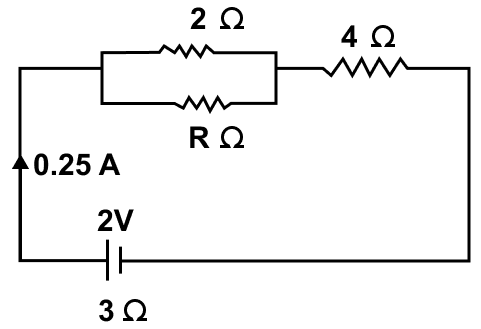(a) Given,

resistor = 4 Ω

I = 0.25 A

the p.d. across the 4 Ω resistor (V) = ?

Using Ohm's law

V = IR

Substituting the values in the formula above we get,

V = 0.25 x 4 = 1 V

Hence, the p.d. across the 4 Ω resistor (V) = 1 V

(b) Given,

internal resistance = 3 Ω

I = 0.25 A

the p.d. across the internal resistance of the cell = ?

Using Ohm's law

V = IR

Substituting the values in the formula above we get,

V = 0.25 x 3 = 0.75 V

Hence, the p.d. across the internal resistance (V) = 0.75 V

(c) Potential difference across R Ω or 2 Ω

V = Vnet - Vacross 4 Ω - Vacross 3 Ω

Hence, we get,

V = 2 - 1 - 0.75 = 0.25 V

(d) The p.d. across resistor of R Ω = 0.25 V

Let the equivalent resistance of the resistors of 2 Ω and R Ω connected in parallel be R'p

$\dfrac{1}{R'_p} = \dfrac{1}{R} + \dfrac{1}{2} \\[0.5em] \Rightarrow \dfrac{1}{R'_p} = \dfrac{2 + R}{2R} \\[0.5em] \Rightarrow R'_p = \dfrac{2R}{2 + R} \\[0.5em]$

Using Ohm's law

V = IR

0.25 = 0.25 x R'p

Substituting the value of R'p from above:

$0.25 = 0.25 \times \Big(\dfrac{2R}{2 + R}\Big) \\[0.5em] \Rightarrow \dfrac{2R}{2 + R} = 1 \\[0.5em] \Rightarrow 2R = 2 + R \\[0.5em] \Rightarrow 2R - R = 2 \\[0.5em] \Rightarrow R = 2 \\[0.5em]$

Hence, value of R = 2 Ω

#### Question 29

Three resistors of 6.0 Ω, 2.0 Ω and 4.0 Ω are joined to an ammeter A and a cell of e.m.f. 6.0 V as shown in figure. Calculate —

(a) the effective resistance of the circuit, and

(b) the reading of ammeter.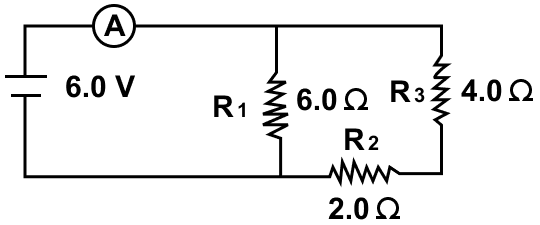In the circuit, there are two parts. In the first part, resistors of 2.0 and 4.0 Ω are connected in series. If the equivalent resistance of this part is Rs then

Rs = 2 + 4 = 6 Ω

In the second part, Rs = 6.0 and resistor of 6.0 Ω are connected in parallel. If the equivalent resistance of this part is Rp then

$\dfrac{1}{R_p} = \dfrac{1}{6} + \dfrac{1}{6} \\[0.5em] \dfrac{1}{R_p} = \dfrac{1 + 1}{6} \\[0.5em] \dfrac{1}{R_p} = \dfrac{2}{6} \\[0.5em] R_p = \dfrac{6}{2} \\[0.5em] R_p = 3.0 Ω \\[0.5em]$

Hence, the effective resistance of the circuit = 3 Ω

(b) The reading of ammeter = ?

R = 3 Ω

V = 6.0 V

Using Ohm's law,

V = IR

Substituting the values in the formula above we get,

6 = I x 3
⇒ I = 6 / 3 = 2 A

Hence, the reading of ammeter = 2 A

#### Question 30

The diagram below in figure shows the arrangement of five different resistances connected to a battery of e.m.f. 1.8 V. Calculate —

(a) the total resistance of the circuit, and

(b) the reading of ammeter A.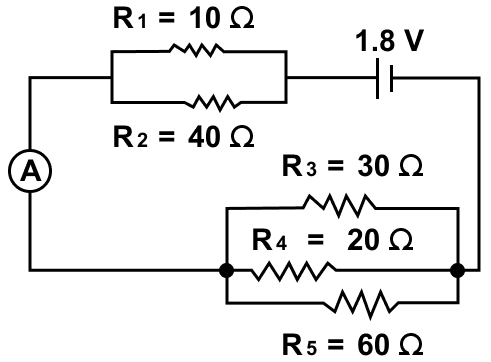(a) In the circuit, there are three parts. In the first part, resistors of 10 Ω and 40 Ω are connected in parallel. If the equivalent resistance of this part is R'p then

$\dfrac{1}{R'_p} = \dfrac{1}{10} + \dfrac{1}{40} \\[0.5em] \dfrac{1}{R'_p} = \dfrac{4 + 1}{40} \\[0.5em] \dfrac{1}{R'_p} = \dfrac{5}{40} \\[0.5em] R'_p = \dfrac{40}{5} \\[0.5em] R'_p = 8 Ω \\[0.5em]$

In the second part, resistors of 30 Ω, 20 Ω and 60 Ω are connected in parallel. If the equivalent resistance of this part is R''p then

$\dfrac{1}{R''_p} = \dfrac{1}{30} + \dfrac{1}{20} + \dfrac{1}{60} \\[0.5em] \dfrac{1}{R''_p} = \dfrac{2 + 3 + 1}{60} \\[0.5em] \dfrac{1}{R''_p} = \dfrac{6}{60} \\[0.5em] R''_p = \dfrac{60}{6} \\[0.5em] R''_p = 10 Ω \\[0.5em]$

In the third part, resistors R'p and R''p are connected in series. If the equivalent resistance of this part is Rs then

$R_s = 8 + 10 \\[0.5em] R_s = 18 Ω \\[0.5em]$

Hence, the total resistance of the circuit = 18 Ω

(b) Given,

e.m.f. = 1.8 V

effective resistance of the circuit = 18 Ω

I = ?

From Ohm's law

V= IR

Substituting the values in the formula above, we get,

$1.8 = I \times 18 \\[0.5em] \Rightarrow I = \dfrac{1.8}{18} \\[0.5em] \Rightarrow I = 0.1 A$

Hence, the reading of ammeter = 0.1 A

#### Question 31

A cell of e.m.f. 2 V and internal resistance 1.2 Ω is connected to an ammeter of resistance 0.8 Ω and two resistors of 4.5 Ω and 9 Ω as shown in figure.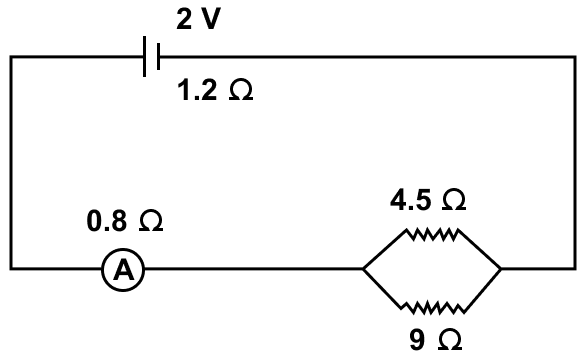Find —

(a) the reading of the ammeter,

(b) the potential difference across the terminals of the cell, and

(c) the potential difference across the 4.5 Ω resistor.

Given,

e.m.f. = 2V

I = ?

In the circuit, there are two parts. In the first part, resistors of 4.5 Ω and 9 Ω are connected in parallel. If the equivalent resistance of this part is Rp then

$\dfrac{1}{R_p} = \dfrac{1}{4.5} + \dfrac{1}{9} \\[0.5em] \dfrac{1}{R_p} = \dfrac{9 + 4.5}{4.5 \times 9} \\[0.5em] \dfrac{1}{R_p} = \dfrac{13.5}{40.5} \\[0.5em] R_p = \dfrac{40.5}{13.5} \\[0.5em] R_p = 3 Ω \\[0.5em]$

In the second part, 1.2 Ω, 0.8 Ω and Rp = 3 Ω are connected in series. If the equivalent resistance of this part is Rs then

$R_s = 1.2 + 0.8 + 3 \\[0.5em] R_s = 5 Ω \\[0.5em]$

Hence, the effective resistance of the circuit = 5 Ω

Using Ohm's law,

V = IR

$2 = I \times 5 \\[0.5em] I = \dfrac{2}{5} \\[0.5em] \Rightarrow I = 0.4 A$

Hence, the reading of the ammeter = 0.4 A

(b) The potential difference across the ends of the cells = ?

ε = 2 V

I = 0.4 A

r = 1.2 Ω

From relation,

Voltage (V) = ε – Ir

Substituting the values in the formula we get,

$V = 2 - (0.4 \times 1.2) \\[0.5em] V = 2 - 0.48 \\[0.5em] V = 1.52 V \\[0.5em]$

Hence, potential difference across the terminals of the cell = 1.52 V

(c) The potential difference across the 4.5 Ω resistor = ?

Current flowing is I = 0.4 A. Now the current I divides in two parts. Let the current in 4.5 Ω resistor be I1 and in 9 Ω resistor be I2.

So I = I1 + I2

and I1 x 4.5 = I2 x 9

On solving,

$I_1 = \dfrac{9}{4.5 + 9} \times I \\[0.5em] I_1 = \dfrac{9}{4.5 + 9} \times 0.4 \\[0.5em] I_1 = 0.2667 \\[0.5em]$

p.d. across the 4.5 Ω resistor

$= I_1 \times 4.5 \\[0.5em] = 0.2667 \times 4.5 \\[0.5em] = 1.2 V$

Alternate Method:

V4.5Ω = Vcell - Vammeter

$V_{4.5Ω} = 1.52 - IR \\[0.5em] V_{4.5Ω} = 1.52 - (0.4 \times 0.8) \\[0.5em] V_{4.5Ω} = 1.52 - 0.32 \\[0.5em] V_{4.5Ω} = 1.2 V$

Hence, p.d. across the 4.5 Ω resistor = 1.2 V

## Exercise 8(C)

#### Question 1

Write an expression for the electrical energy spent in flow of current through an electrical appliance in terms of current, resistance and time.

The expression for electrical energy spent in flow of current through an electrical appliance in terms of current, resistance and time is:

W = I2Rt

#### Question 2

Write an expression for the electrical power spent in flow of current through a conductor in terms of (a) resistance and potential difference, (b) current and resistance.

(a) Expression for electrical power spent in flow of current through a conductor in terms of resistance and potential difference is —

$W = \dfrac{V^2}{R}$

(b) Expression for electrical power spent in flow of current through a conductor in terms of current and resistance is —

$W = I^2R$

#### Question 3

Electrical power P is given by the expression P = $\dfrac{(Q × V)}{\text{time}}$.

(a) What do the symbols Q and V represent?

(b) Express the power P in terms of current and resistance explaining the meaning of symbols used there in.

(a) In the expression,

$P = \dfrac{Q \times V}{time}$

Symbol Q represents charge and the symbol V represents voltage.

(b) The expression for power P in terms of current and resistance is:

$P = I^2R$

where,

I represents current and

R represents resistance.

#### Question 4

Name the S.I. unit of electrical energy. How is it related to Wh?

The S.I. unit of electrical energy is Joule.

The relation between Wh and Joule is as follows —

1 Wh = 3600 J

#### Question 5

Explain the meaning of the statement 'the power of an appliance is 100 W'.

The statement 'the power of an appliance is 100 W' means that 100 J of electrical energy is consumed by the appliance in 1 second.

#### Question 6

State the S.I. unit of electrical power.

The S.I. unit of electrical power is volt x ampere (VA) or watt (W) or J s-1

One watt is the electric power consumed when a current of 1 ampere flows through a circuit having a potential difference of 1 volt.

#### Question 7

(i) State and define the household unit of electricity.

(ii) What is the voltage of the electricity that is generally supplied to a house?

(iii) What is consumed while using different electrical appliances, for which electricity bills are paid?

(i) The household unit of electricity is Kilowatt hour (kWh).

One kilowatt-hour (kWh) is the electrical energy consumed by an electrical appliance of power 1 kilowatt when it is used for 1 hour.

(ii) The voltage of the electricity that is generally supplied to a house is 220 volt.

(iii) The electrical energy is consumed by various appliances in our houses (or industries) and it's cost is paid to the electrical company.

#### Question 8

Name the physical quantity which is measured in (i) kW, (ii) kWh. (iii) Wh

(i) The physical quantity measured in kW is electrical power.

(ii) The physical quantity measured in kWh is electrical energy. kWh is commercial unit of electrical energy.

(iii) The physical quantity measured in Wh is electrical energy. Wh is commercial unit of electrical energy.

#### Question 9

Define the term kilowatt-hour and state it's value in S.I. unit.

One kilowatt-hour (kwh) is the electrical energy consumed by an electrical appliance of power 1 kilowatt when it is used for 1 hour.

Value of kilowatt-hour (kWh) in S.I. unit is —

1 kWh = 3.6 × 106 J

#### Question 10

How do kilowatt and kilowatt-hour differ?

Kilowatt is the unit of electrical power. i.e., one kilowatt is the electric power consumed when a current of 1 ampere flows through a circuit having a potential difference of 1 volt.

Kilowatt-hour is the unit of electrical energy i.e., One kilowatt - hour (kwh) is the electrical energy consumed by an electrical appliance of power 1 kilowatt when it is used for 1 hour.

#### Question 11

Complete the following —

$\text{(a) 1 kWh} = \dfrac{1 \text{ volt} \times 1 \text{ ampere} \times \text{……} }{1000} \\[2em] \text{(b) 1 kWh} = \text{…… J}$

$\text{(a) 1 kWh} = \dfrac{1 \text{ volt} \times 1 \text{ ampere} \times \boldsymbol{1 \space hour} }{1000} \\[2em] \text{(b) 1 kWh} = \boldsymbol{3.6 \times 10^6 J}$

#### Question 12

What do you mean by power rating of an electrical appliance? How do you use it to calculate (a) the resistance of the appliance and (b) the safe limit of the current in it, while in use ?

An electrical appliance such as electric heater, geyser etc is rated with it's power and voltage.

For example, an electric bulb is rated as 100 W - 220 V. It means that if the bulb is lighted on a 220 V supply, the electric power consumed by it is 100 W (i.e., 100 J of electric energy is consumed by the bulb in 1 s).

(a) Resistance of element of appliance while in use is —

$R = \dfrac{V^2}{P}$

(b) The safe limit of current in it, while in use is —

$I = \dfrac{P}{V}$

If current exceeds this value, the power supplied at voltage V will exceed the rated power of the appliance and the appliance may get damaged. So this value of current is called the safe current which can flow through the appliance at voltage V.

#### Question 13

An electric bulb is rated '100 W, 250 V'. What information does this convey ?

When an electric bulb is rated '100 W, 250 V' it means that if the bulb is lighted on a 250 V supply, the electric power consumed by it is 100 W (i.e., 100 J of electrical energy is consumed by the bulb in 1 s).

#### Question 14

List the names of three electrical gadgets used in your house. Write their power, voltage rating and approximate time for which each one is used in a day. Hence find the electrical energy consumed by each in a month of 30 days.

AppliancePower
(in kilowatt)
Voltage
(V)
Time
(t)
Electrical energy
(E = P × t)
in (kWh)
Fluorescent tube0.0422060.24
Television set0.1222030.36
Room heater122022

(i) Energy consumed by fluorescent tube in one day = 0.24 kWh

Hence, energy consumed in 30 days = 0.24 x 30 = 7.2 kWh.

(ii) Energy consumed by television set in one day = 0.36 kWh

Hence, energy consumed in 30 days = 0.36 x 30 = 10.8 kWh.

(iii) Energy consumed by room heater in one day = 2 kWh

Hence, energy consumed in 30 days = 2 x 30 = 60 kWh.

#### Question 15

Two lamps, one rated 220 V, 50 W and the other rated 220 V, 100 W, are connected in series with mains of voltage 220 V. Explain why does the 50 W lamp consume more power.

Resistance of lamp A (rating 220 V, 50 W) is —

$R_A = \dfrac{V^2}{P_A}$

Substituting the values in the formula above we get,

$R_A = \dfrac{220^2}{50} \\[0.5em] \Rightarrow R_A = 968 Ω$

Resistance of lamp B (rating 220 V, 100 W) is —

$R_B = \dfrac{V^2}{P_B}$

Substituting the values in the formula above we get,

$R_B = \dfrac{220^2}{100} \\[0.5em] \Rightarrow R_B = 484 Ω$

From formula, we get, P = I2 R

As we observe that RA > RB and same current is flowing in both the lamps as both are connected in series therefore PA > PB. Hence, 50 W lamp consumes more power than 100 W lamp.

#### Question 16

Name the factors on which the heat produced in a wire depends when current is passed in it, and state how does it depend on the factors stated by you.

The amount of heat produced in a wire on passing current through it, depends on the following three factors.

1. The amount of current passing through the wire — The amount of heat H produced in a wire is directly proportional to the square of current I passing through the wire, i.e., H ∝ I2
2. The resistance of wire — The amount of heat H produced in the wire is directly proportional to the resistance R of the wire, i.e., H ∝ R.
3. The time for which current is passed in the wire — The amount of heat H produced in a wire is directly proportional to the time t for which current is passed in the wire i.e., H ∝ t

## Multiple Choice Type

#### Question 1

When a current I flows through a resistance R for time t, the electrical energy spent is —

1. IRt
2. I2Rt ✓
3. IR2t
4. $\dfrac{I^2R}{t}$

The electrical energy spent when a current I flows through a resistance R for time t is I2Rt.

#### Question 2

An electrical appliance has a rating 100 W, 120 V. The resistance of element of appliance when in use is —

1. 1.2 Ω
2. 144 Ω ✓
3. 120 Ω
4. 100 Ω

Resistance of an electrical appliance (rating 100 W, 120 V) is —

$R_A = \dfrac{V^2}{P_A}$

Substituting the values in the formula above we get,

$R_A = \dfrac{120^2}{100} \\[0.5em] \Rightarrow R_A = 144 Ω$

Hence, resistance of an electrical appliance = 144 Ω

## Numericals

#### Question 1

An electric bulb of resistance 500 Ω draws current 0.4 A from the source. Calculate: (a) the power of bulb and (b) the potential difference at it's end.

(a) Given,

Resistance (R) = 500 Ω

Current (I) = 0.4 A

Power = ?

From Ohm's law:

V = IR

Substituting the values in the formula above, we get,

V = 0.4 x 500 = 200 V

Power (P) = VI

Substituting the values in the power formula we get,

P = 200 x 0.4 = 80 W

Hence, the power of bulb = 80 W

(b) The potential difference at it's end = 200 V

#### Question 2

A current of 2 A is passed through a coil of resistance 75 Ω for 2 minutes. (a) How much heat energy is produced? (b) How much charge is passed through the resistance ?

(a) Given,

Current (I) = 2 A

Resistance (R) = 75 Ω

Time (t) = 2 min = 120 s

Heat produced = ?

Using

H = I2Rt

Substituting the values in the formula above, we get,

$H = 2^2 \times 75 \times 120 \\[0.5em] \Rightarrow H = 36000 \text{J}$

Hence, heat energy produced = 36000 J

(b) Charge passed through the resistance = ?

From relation,

Q = It

Substituting the values in the formula above we get,

$Q = 2 \times 120 \\[0.5em] \Rightarrow Q = 240 \text{ C}$

Hence, charge passed through the resistance = 240 C

#### Question 3

Calculate the current through a 60 W lamp rated for 250 V. If the line voltage falls to 200 V, how is power consumed by the lamp affected?

Given,

Power (P) = 60 W

Voltage (V) = 250 V

We know that,

Power (P) = VI

Substituting the values in the formula above, we get,

$60 = 250 \times I \\[0.5em] \Rightarrow I = \dfrac{60}{250} \\[0.5em] \Rightarrow I = 0.24 A$

Hence, current through the lamp = 0.24 A

Power consumed when voltage is 200 V = ?

Resistance of lamp (R) = $\dfrac{V^2}{P}$

Substituting the values in the formula, we get,

$R = \dfrac{250^2}{60} \\[0.5em] \Rightarrow R = 1041.6 Ω$

From relation,

P = $\dfrac{V^2}{R}$

Substituting the values in the formula, we get,

$P = \dfrac{200^2}{1041.6} \\[0.5em] \Rightarrow P = 38.4 W$

Hence, power consumed reduces to 38.4 W

#### Question 4

An electric bulb is rated '100 W, 250 V'. How much current will the bulb draw if connected to a 250 V supply ?

Given,

Power (P) = 100 W

Voltage (V) = 250 V

From relation,

Power (P) = VI

Substituting the values in the formula above, we get,

$100 = 250 \times I \\[0.5em] \Rightarrow I = \dfrac{100}{250} \\[0.5em] \Rightarrow I = 0.4 \text {A}$

Hence, current drawn = 0.4 A

#### Question 5

An electric bulb is rated '220 V, 100 W'. (a) What is it's resistance? (b) What safe current can be passed through it?

(a) Given,

P = 100 W

V = 220 volt

We know that,

Power P = $\dfrac{V^2}{R}$

Substituting the values in the formula above, we get,

$100 = \dfrac{220^2}{R} \\[0.5em] \Rightarrow R = \dfrac{220^2}{100} \\[0.5em] \Rightarrow R = 484 Ω \\[0.5em]$

Hence, resistance of electric bulb = 484 Ω

(b) From relation P = VI

Safe current I = $\dfrac{P}{V}$

Substituting the value we get,

$I = \dfrac{100}{220} \\[0.5em] \Rightarrow I = 0.45 A$

Hence, safe current limit = = 0.45 A

#### Question 6

A bulb of power 40 W is used for 12.5 h each day for 30 days. Calculate the electrical energy consumed.

Given,

Power (P) = 40 W

time (t) = 12.5 h for 30 days

As energy consumed (E) = P × t

$E = 40 \times 12.5 \\[0.5em] \Rightarrow E = 500 \text { Wh}$

Hence, electrical energy consumed in one day = 500 Wh

Electrical energy consumed for in 30 days = ?

$E = 500 \times 30 \\[0.5em] E = 15000 \text { Wh} \\[0.5em] E = 15 \text { kWh} \\[0.5em]$

Hence, electrical energy consumed in 30 days = 15 kWh

#### Question 7

An electric press is rated '750 W, 230 V'. Calculate the electrical energy consumed by the press in 16 hours.

Given,

Power (P) = 750 W

time (t) = 16 h

As energy consumed (E) = P × t

$E = 750 \times 16 \\[0.5em] \Rightarrow E = 12000 \text { Wh} \\[0.5em] \Rightarrow E = 12 \text { kWh} \\[0.5em]$

Hence, electrical energy consumed in one day = 12 kWh

#### Question 8

An electrical appliance having a resistance of 200 Ω is operated at 200 V. Calculate the energy consumed by the appliance in 5 minutes (i) in joule, (ii) in kWh

(i) Given,

Resistance (R) = 200 Ω

Voltage (V) = 200 volt

Time (t) = 5 min = 300 sec

As we know,

Energy (E) = $\dfrac{V^2t}{R}$

Substituting the values in the formula above we get,

$E = \dfrac{200^2 \times 300}{200} \\[0.5em] \Rightarrow E = 60,000 \text{ J}$

Hence, energy consumed = 60,000 J

(ii) In kWh = ?

As we know,

$1 \text{kWh} = 3.6 \times 10^6 \text{J} \\[0.5em] 1 \text{ J} = \dfrac{1}{3.6 \times 10^6} \text{kWh} \\[0.5em] 60,000 \text{ J} = \dfrac{60,000}{3.6 \times 10^6} \\[0.5em] \Rightarrow 60,000 \text{ J}= 0.0167 \text { kWh} \\[0.5em]$

Hence, energy consumed = 0.0167 kWh

#### Question 9

A bulb rated 12 V, 24 W operates on a 12 volt battery for 20 minutes. Calculate —

(i) the current flowing through it, and

(ii) the energy consumed.

(i) Given,

Voltage (V) = 12 V

Power (P) = 24 W

Current (I) = ?

From relation,

Power (P) = VI

Substituting the values in the formula above, we get,

$24 = 12 \times I \\[0.5em] \Rightarrow I = \dfrac{24}{12} \\[0.5em] \Rightarrow I = 2 A$

Hence, the current flowing through it = 2 A

(ii) Energy (E) = P × t

Time (t) = 20 min = 1200 sec

$E = 24 \times 1200 \\[0.5em] E = 28,800 J$

Hence, energy consumed = 28,800 J

#### Question 10

A current of 0.2 A flows through a wire whose ends are at a potential difference of 15 V. Calculate —

(i) the resistance of the wire, and

(ii) the heat energy produced in 1 minute.

(a) Given,

Current (I) = 0.2 A

Potential difference (V) = 15 V

From Ohm's law

V = IR

Substituting the values in the formula above, we get,

$15 = 0.2 \times R \\[0.5em] \Rightarrow R = \dfrac{15}{0.2} \\[0.5em] \Rightarrow R = \dfrac{150}{2} \\[0.5em] \Rightarrow R = 75 Ω$

Hence, the resistance of the wire = 75 Ω

(ii) Given,

Time (t) = 60 sec

heat energy produced in 1 minute = ?

From relation,

Heat energy (H) = I2Rt

Substituting the values in the formula above, we get,

H = 0.22 x 75 x 60 = 180 J

#### Question 11

What is the resistance, under normal working conditions, of an electric lamp rated at '240 V, 60 W'? If two such lamps are connected in series across a 240 V mains supply, explain why each one appears less bright.

Given,

Voltage (V) = 240 V

Power (P) = 60 W

Resistance (R) = ?

From relation

$P = \dfrac{V^2}{R} \\[0.5em]$

We get,

$60 = \dfrac{240^2}{R} \\[0.5em] \Rightarrow R = \dfrac{240^2}{60} \\[0.5em] \Rightarrow R = 960 Ω$

Hence, resistance of the electric lamp = 960 Ω

From the relation for current through the element, we know,

$I = \dfrac{P}{V}$

Substituting the values in the formula above, we get,

$I = \dfrac{60}{240} \\[0.5em] \Rightarrow I = 0.25 A$

When one lamp is connected across the mains, it draws 0.25 A current. If two such lamps are connected in series across the mains, current through each bulb becomes —

$\dfrac{240 V}{(960 + 960) Ω} \\[0.5em] = 0.125 A$

i.e., current is halved. Hence, heating (= I2Rt) in each bulb becomes one fourth, so each bulb appears less bright.

#### Question 12

Two bulbs are rated '60 W, 220 V' and '60 W, 110 V' respectively. Calculate the ratio of their resistances.

Given,

Voltage (V1) = 220 V

Voltage (V2) = 110 V

P = 60 W (both bulbs have same power)

Resistance (R) = ?

From relation

$P = \dfrac{V^2}{R} \\[0.5em]$

Case 1:

$60 = \dfrac{220^2}{R} \\[0.5em] \Rightarrow R_1 = \dfrac{220^2}{60} \\[0.5em]$

Case 2:

$60 = \dfrac{110^2}{R} \\[0.5em] \Rightarrow R_2 = \dfrac{110^2}{60} \\[0.5em]$

Ratio between the two is —

$R_1 : R_2 = \dfrac{220^2}{60} : \dfrac{110^2}{60} \\[0.5em] R_1 : R_2 = \dfrac{220^2}{110^2} \\[0.5em] R_1 : R_2 = \dfrac{2^2}{1^2} \\[0.5em] R_1 : R_2 = \dfrac{4}{1} \\[0.5em]$

Hence, ratio of resistances of the two bulbs is = 4 : 1

#### Question 13

An electric bulb is rated '250 W, 230 V'. Calculate

(i) the energy consumed in one hour, and

(ii) the time in which the bulb will consume 1.0 kWh energy when connected to 230 V mains?

(i) Given,

Power (P) = 250 W

Voltage (V) = 230 V

Time (t) = 1 h = 3600 sec

Energy (E) = ?

From relation,

E = P × t

Substituting the values in the formula above, we get,

E = 250 x 3600 = 9 x 105 J

Hence, energy consumed in one hour = 9 x 105 J

(ii) Let t be the time in which the bulb will consume 1.0 kWh energy when connected to 230 V mains

P = 1.0 kWh = 1000 Wh

Substituting the value in the formula, we get,

1000 = 250 x t
⇒ t = 1000 / 250 = 4 h

Hence, time = 4 h

#### Question 14

Three heaters each rated 250 W, 100 V are connected in parallel to a 100 V supply.

Calculate —

(i) The total current taken from the supply,

(ii) the resistance of each heater, and

(iii) the energy supplied in kWh to the three heaters in 5 hours.

(i) Given,

Power (P) = 250 W

Voltage (V) = 100 V

Current through each heater (I) = ?

As P = VI

Substituting the values in the formula above we get,

250 = 100 x I
⇒ I = 250 / 100 = 2.5 A

Hence, current through each heater = 2.5 A

Therefore, current for three heaters =
I = 3 x 2.5 = 7.5 A

Hence, total current taken from supply = 7.5 A

(ii) Resistance for each heater (R) = ?

Since, V = IR

Substituting the values in the formula above we get,

100 = 2.5 x R
⇒ R = 100 / 2.5 = 40 Ω

Hence, resistance of each heater = 40 Ω

(iii) Energy supplied to three heater in 5 h = ?

Energy (E) = P × t

Substituting the values in the formula above, we get,

E = 250 x 5 = 1250 Wh = 1.25 kWh

Hence, Energy for three heaters = 3 × 1.25 = 3.75 kWh

Hence, energy supplied to the three heaters = 3.75 kWh

#### Question 15

A bulb is connected to a battery of p.d. 4 V and internal resistance 2.5 ohm. A steady current of 0.5 A flows through the circuit. Calculate —

(i) the total energy supplied by the battery in 10 minutes,

(ii) the resistance of the bulb, and

(iii) the energy dissipated in the bulb in 10 minutes.

(i) Given,

Voltage (V) = 4 V

Internal resistance = 2.5 Ω

Current (I) = 0.5 A

time (t) = 10 min = 600 sec

Energy supplied by the battery (E) = V x I x t

Substituting the values in the formula, we get,

E = 4 x 0.5 x 600 = 1200 J

Hence, total energy supplied = 1200 J

(ii) resistance of the bulb = ?

From relation V = I (R + r)

We get,

4 = 0.5 (R + 2.5)
⇒ 4 = (0.5 R) + (0.5 x 2.5)
⇒ 4 = (0.5 R) + 1.25
⇒ 0.5 R = 4 - 1.25
⇒ R = 2.75 / 0.5 = 5.5 Ω

Hence, resistance of the bulb = 5.5 Ω

(iii) Energy dissipated in the bulb in 10 min = ?

From relation

E = I2Rt

Substituting the values in the formula above, we get,

E = 0.5 x 0.5 x 5.5 x 600 = 825 J

Hence, energy dissipated = 825 J

#### Question 16

Two resistors A and B of resistance 4 Ω and 6 Ω respectively are connected in parallel. The combination is connected across a 6 volt battery of negligible resistance. Calculate : (i) the power supplied by the battery, (ii) the power dissipated in each resistor.

(i) Given,

Resistance, RA = 4 Ω

Resistance, RB = 6 Ω

Let equivalent resistance of the two resistors connected in parallel be RP

$\dfrac{1}{R_P} = \dfrac{1}{4} + \dfrac{1}{6} \\[0.5em] \dfrac{1}{R_P} = \dfrac{3 + 2}{12} \\[0.5em] \dfrac{1}{R_P} = \dfrac{5}{12} \\[0.5em] R_P = \dfrac{12}{5} \\[0.5em] R_P = 2.4 Ω \\[0.5em]$

Hence, equivalent resistance = 2.4 Ω

From relation

$P = \dfrac{V^2}{R} \\[0.5em]$

We get,

$P = \dfrac{6^2}{2.4} \\[0.5em] \Rightarrow P = 15 W$

Hence, power supplied = 15 W

(ii) Power dissipation across each resistor = ?

From relation, P = VI

Current across A (4 Ω) resistor —

IA = 6 / 4 = 1.5 A

Power dissipation across A = VIA

Substituting we get,

P = 6 x 1.5 = 9 W

Similarly,

Current across B ( 6 Ω) resistor —

IB = 6 / 6 = 1 A

Power dissipation across B resistor = VIB

Substituting the values in the formula above, we get,

P = 6 x 1 = 6 W

Hence, power dissipated across A = 9 W and across B = 6 W

#### Question 17

A battery of e.m.f. 15 V and internal resistance 2 Ω is connected to two resistors of resistances 4 Ω and 6 Ω joined in series. Find the electrical energy spent per minute in 6 Ω resistor.

(i) Given,

e.m.f. (V) = 15 V

Internal resistance = 2 Ω

Resistors are R1 = 4 Ω and R2 = 6 Ω

Electrical energy = ?

As the battery and resistors are connected in series, equivalent resistance is given by

R = 2 + 4 + 6 = 12 Ω

From Ohm's law —

V = IR

Substituting the values in the formula above, we get,

15 = I x 12
⇒ I = 15 / 12 = 1.25 A

Hence, current in circuit = 1.25 A

Now,

Voltage across 6 Ω = IR = 1.25 × 6 = 7.50 V

Hence, Voltage across 6 Ω = 7.5 V

Time (t) = 1 min = 60 sec

We know, E = $\dfrac{V^2 \times t}{R}$

Substituting the values in the formula above, we get,

$E = \dfrac{7.5^2 \times 60}{6} \\[0.5em] E = 7.5^2 \times 10 \\[0.5em] \Rightarrow E = 562.5 J$

Hence, energy spent across the 6 Ω resistor = 562.5 J

#### Question 18

Water in an electric kettle connected to a 220 V supply takes 5 minutes to reach it's boiling point. How long will it take if the supply voltage falls to 200 V?

Given,

V = 220 V

time (t) = 5 min = 300 sec

From relation

$P = \dfrac{V^2}{R} \\[0.5em]$

and

Heat produced (H) —

$H = P \times t \\[0.5em] \Rightarrow H = (\dfrac{V^2}{R}) \times t \\[0.5em]$

Case 1 for V = 220 V

$H = (\dfrac{220^2}{R}) \times 300 \\[0.5em]$

[Equation 1]

Case 2 for V = 200 V

$H = (\dfrac{200^2}{R}) \times t \\[0.5em]$

[Equation 2]

Equating 1 and 2 we get,

$(\dfrac{220^2}{R}) \times 300 = (\dfrac{200^2}{R}) \times t \\[0.5em] \Rightarrow t = (\dfrac{220^2}{200^2}) \times 300 \\[0.5em] \Rightarrow t = 363 \text { sec} \\[0.5em] \Rightarrow t = 6.05 \text { min} \\[0.5em]$